Скачать презентацию Constraint Satisfaction Problems Chapter 5 CS 370

a6f070257339a662c6044ee7c132913e.ppt

• Количество слайдов: 84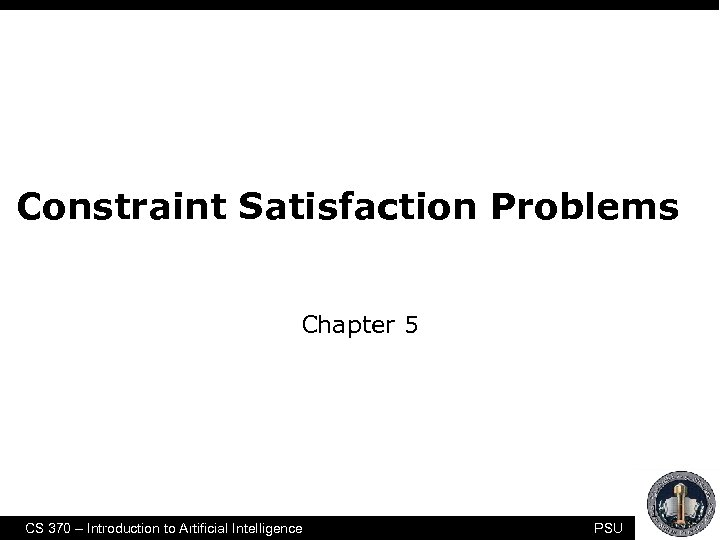Constraint Satisfaction Problems Chapter 5 CS 370 – Introduction to Artificial Intelligence PSU 1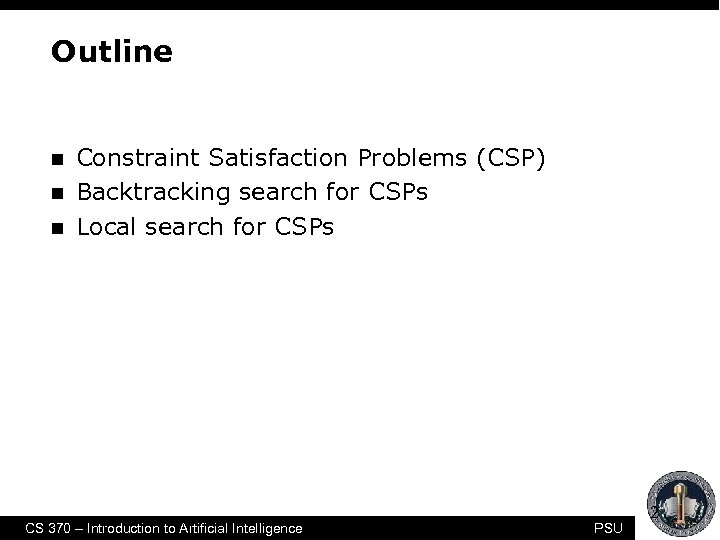Outline n n n Constraint Satisfaction Problems (CSP) Backtracking search for CSPs Local search for CSPs CS 370 – Introduction to Artificial Intelligence PSU 2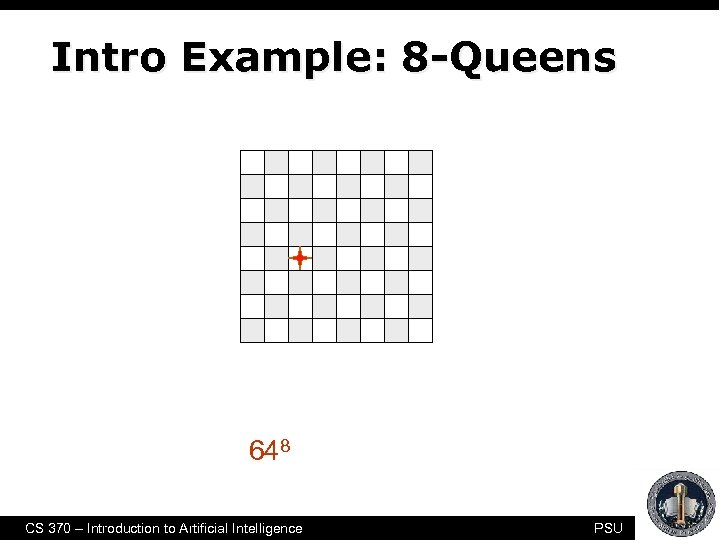Intro Example: 8 -Queens • Purely generate-and-test • The “search” tree is only used to enumerate all possible 648 combinations CS 370 – Introduction to Artificial Intelligence PSU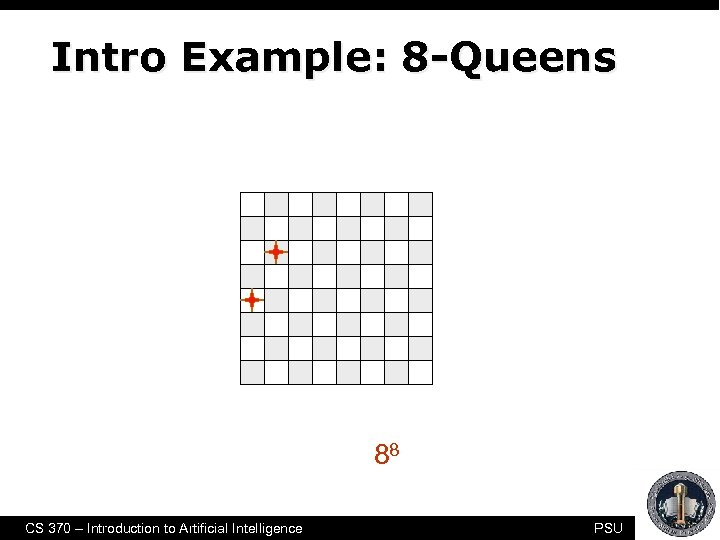Intro Example: 8 -Queens Another form of generate-and-test, with no redundancies “only” 88 combinations CS 370 – Introduction to Artificial Intelligence PSU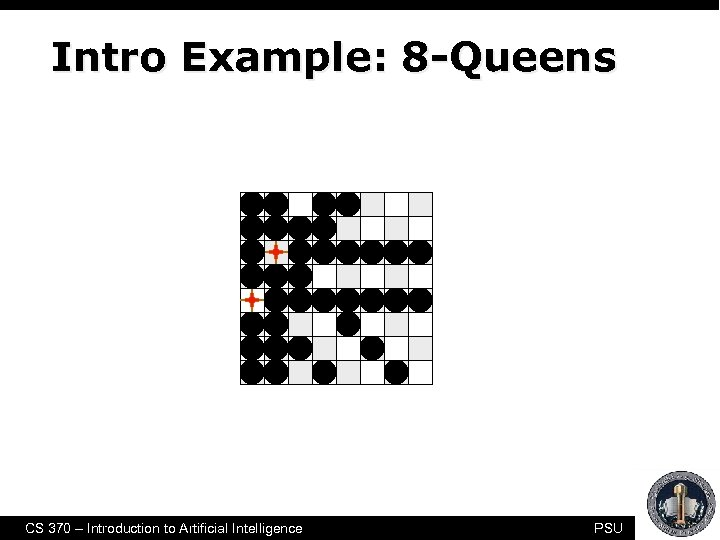Intro Example: 8 -Queens CS 370 – Introduction to Artificial Intelligence PSU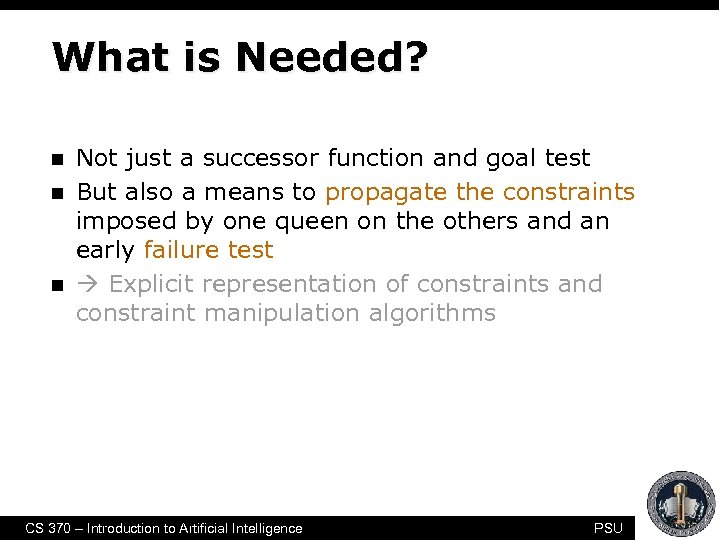What is Needed? n n n Not just a successor function and goal test But also a means to propagate the constraints imposed by one queen on the others and an early failure test Explicit representation of constraints and constraint manipulation algorithms CS 370 – Introduction to Artificial Intelligence PSU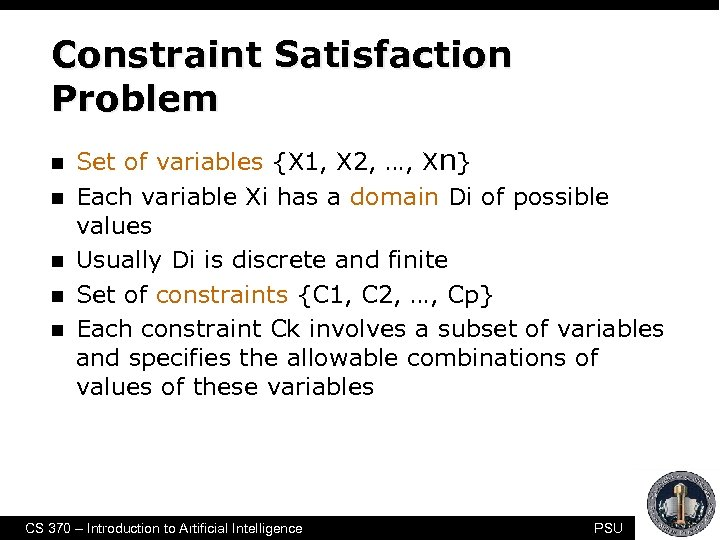Constraint Satisfaction Problem n n n Set of variables {X 1, X 2, …, Xn} Each variable Xi has a domain Di of possible values Usually Di is discrete and finite Set of constraints {C 1, C 2, …, Cp} Each constraint Ck involves a subset of variables and specifies the allowable combinations of values of these variables CS 370 – Introduction to Artificial Intelligence PSU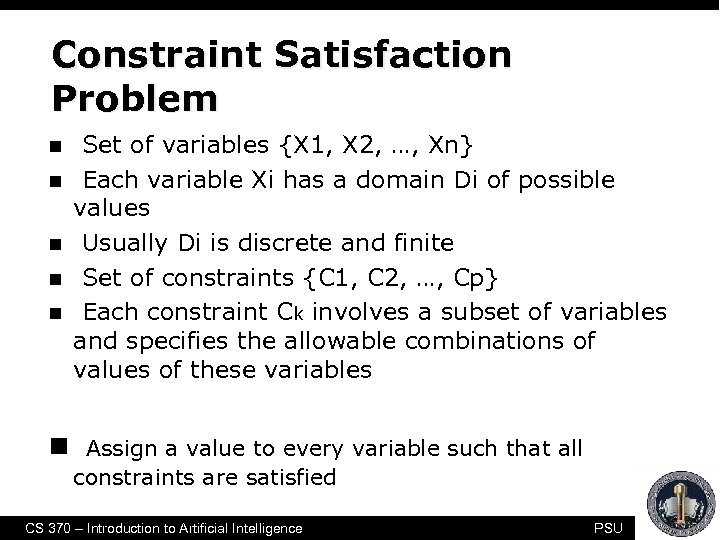Constraint Satisfaction Problem n n n Set of variables {X 1, X 2, …, Xn} Each variable Xi has a domain Di of possible values Usually Di is discrete and finite Set of constraints {C 1, C 2, …, Cp} Each constraint Ck involves a subset of variables and specifies the allowable combinations of values of these variables Assign a value to every variable such that all constraints are satisfied CS 370 – Introduction to Artificial Intelligence PSU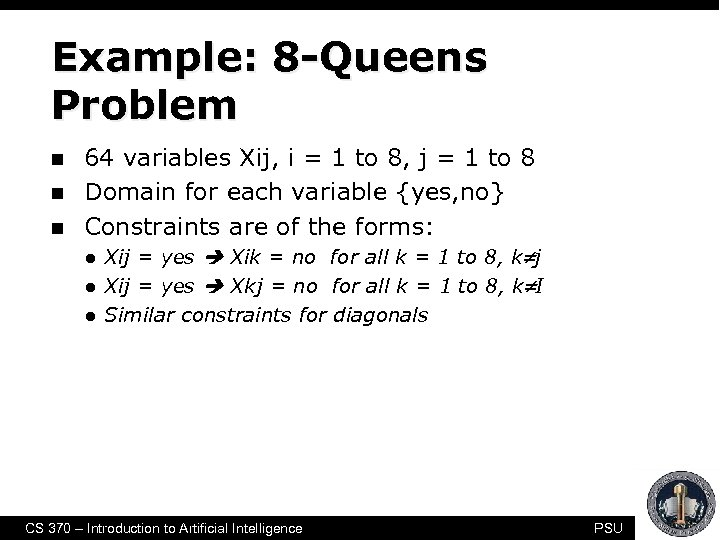Example: 8 -Queens Problem n n n 64 variables Xij, i = 1 to 8, j = 1 to 8 Domain for each variable {yes, no} Constraints are of the forms: l l l Xij = yes Xik = no for all k = 1 to 8, k j Xij = yes Xkj = no for all k = 1 to 8, k I Similar constraints for diagonals CS 370 – Introduction to Artificial Intelligence PSU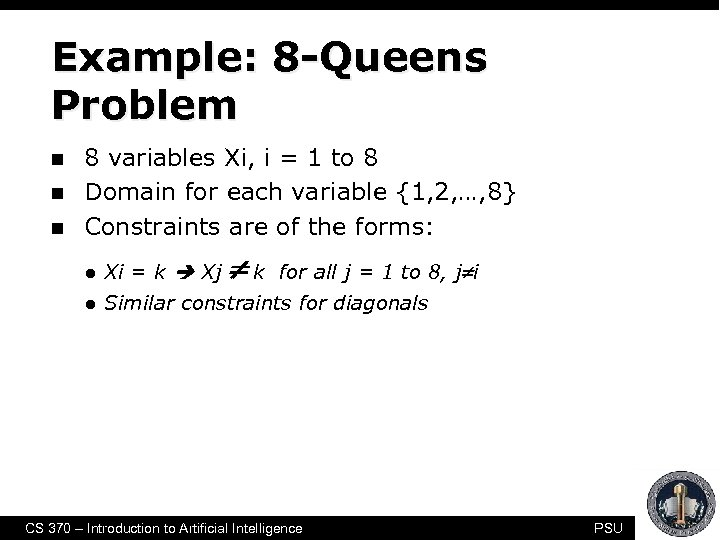Example: 8 -Queens Problem n n n 8 variables Xi, i = 1 to 8 Domain for each variable {1, 2, …, 8} Constraints are of the forms: k for all j = 1 to 8, j i l Xi = k Xj l Similar constraints for diagonals CS 370 – Introduction to Artificial Intelligence PSU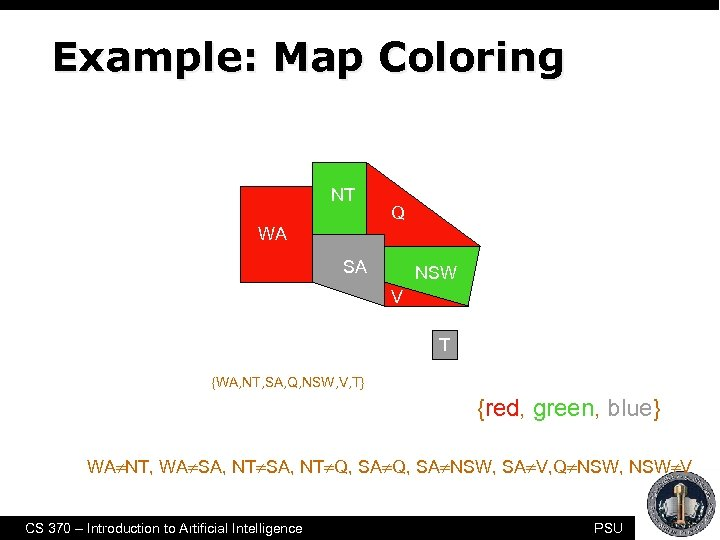Example: Map Coloring NT Q WA SA NSW V T • 7 variables {WA, NT, SA, Q, NSW, V, T} • Each variable has the same domain {red, green, blue} • No two adjacent variables have the same value: WA NT, WA SA, NT Q, SA NSW, SA V, Q NSW, NSW V CS 370 – Introduction to Artificial Intelligence PSU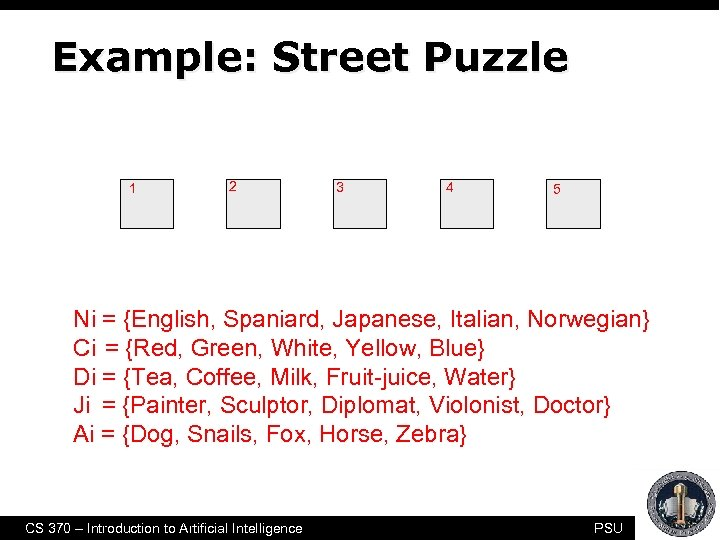Example: Street Puzzle 1 2 3 4 5 Ni = {English, Spaniard, Japanese, Italian, Norwegian} Ci = {Red, Green, White, Yellow, Blue} Di = {Tea, Coffee, Milk, Fruit-juice, Water} Ji = {Painter, Sculptor, Diplomat, Violonist, Doctor} Ai = {Dog, Snails, Fox, Horse, Zebra} CS 370 – Introduction to Artificial Intelligence PSU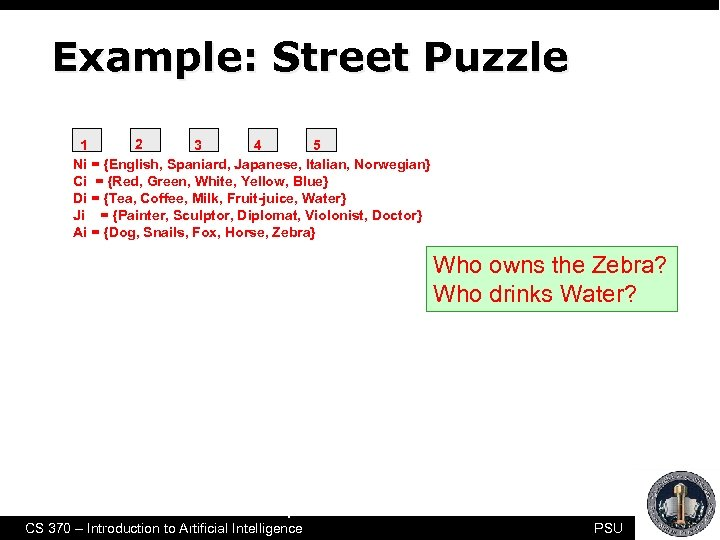Example: Street Puzzle 2 3 4 1 5 Ni = {English, Spaniard, Japanese, Italian, Norwegian} Ci = {Red, Green, White, Yellow, Blue} Di = {Tea, Coffee, Milk, Fruit-juice, Water} Ji = {Painter, Sculptor, Diplomat, Violonist, Doctor} Ai = {Dog, Snails, Fox, Horse, Zebra} The Englishman lives in the Red house Who The Spaniard has a Dog Who The Japanese is a Painter The Italian drinks Tea The Norwegian lives in the first house on the left The owner of the Green house drinks Coffee The Green house is on the right of the White house The Sculptor breeds Snails The Diplomat lives in the Yellow house The owner of the middle house drinks Milk The Norwegian lives next door to the Blue house The Violonist drinks Fruit juice The Fox is in the house next to the Doctor’s The Horse is next to the Diplomat’s CS 370 – Introduction to Artificial Intelligence owns the Zebra? drinks Water? PSU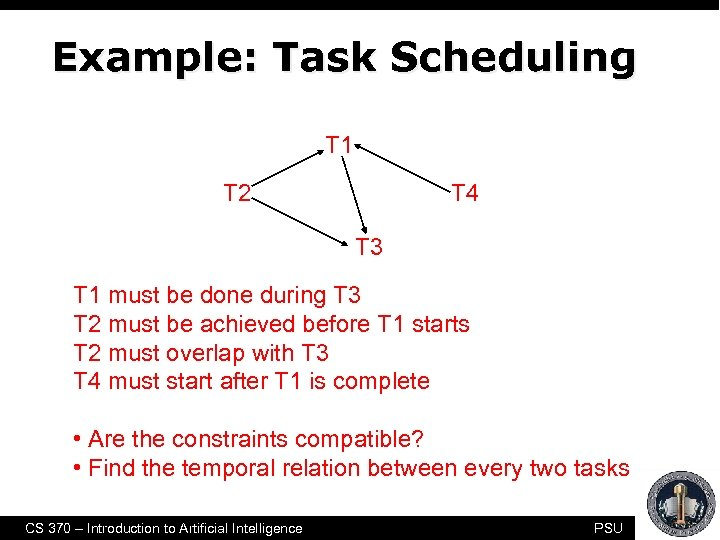Example: Task Scheduling T 1 T 2 T 4 T 3 T 1 must be done during T 3 T 2 must be achieved before T 1 starts T 2 must overlap with T 3 T 4 must start after T 1 is complete • Are the constraints compatible? • Find the temporal relation between every two tasks CS 370 – Introduction to Artificial Intelligence PSU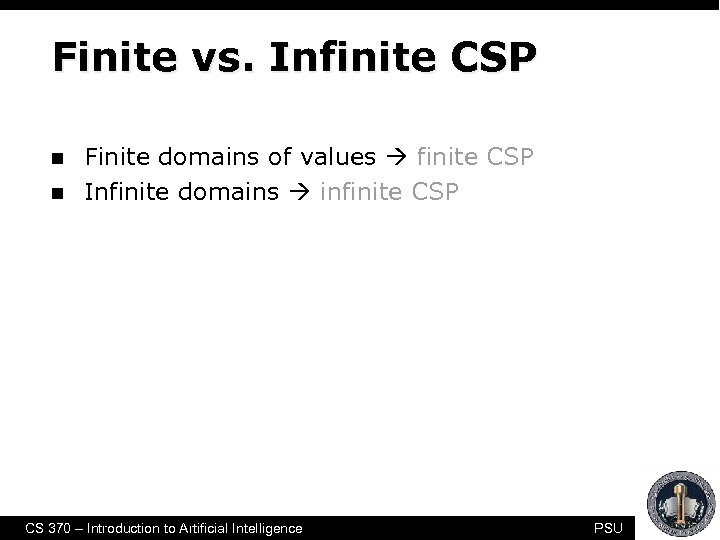Finite vs. Infinite CSP n n Finite domains of values finite CSP Infinite domains infinite CSP CS 370 – Introduction to Artificial Intelligence PSU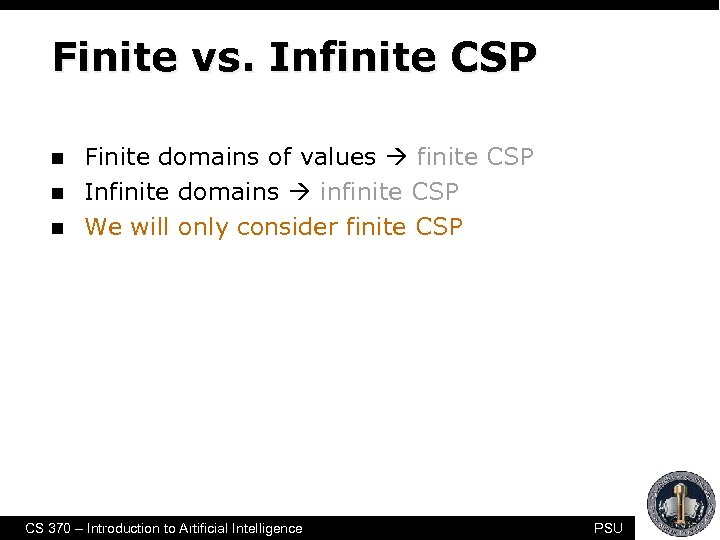Finite vs. Infinite CSP n n n Finite domains of values finite CSP Infinite domains infinite CSP We will only consider finite CSP CS 370 – Introduction to Artificial Intelligence PSU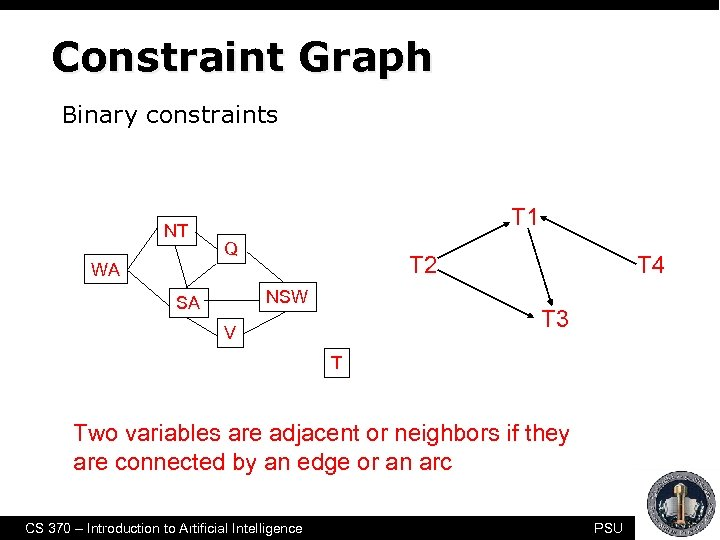Constraint Graph Binary constraints NT T 1 Q T 2 WA NSW SA T 4 T 3 V T Two variables are adjacent or neighbors if they are connected by an edge or an arc CS 370 – Introduction to Artificial Intelligence PSU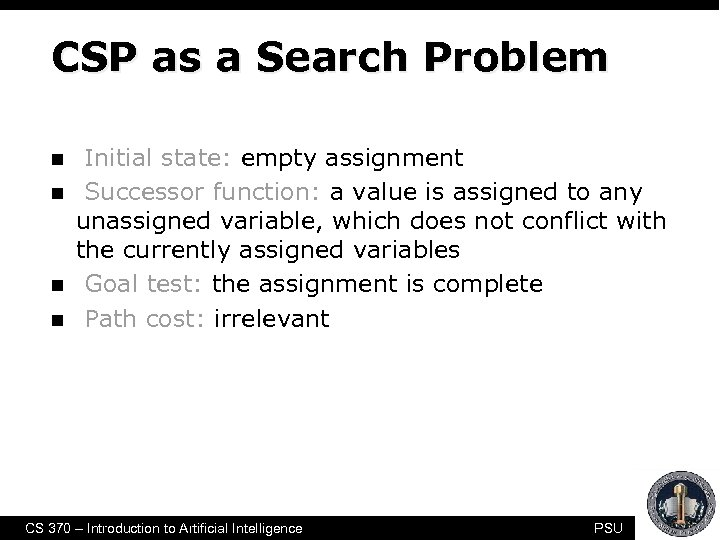CSP as a Search Problem n n Initial state: empty assignment Successor function: a value is assigned to any unassigned variable, which does not conflict with the currently assigned variables Goal test: the assignment is complete Path cost: irrelevant CS 370 – Introduction to Artificial Intelligence PSU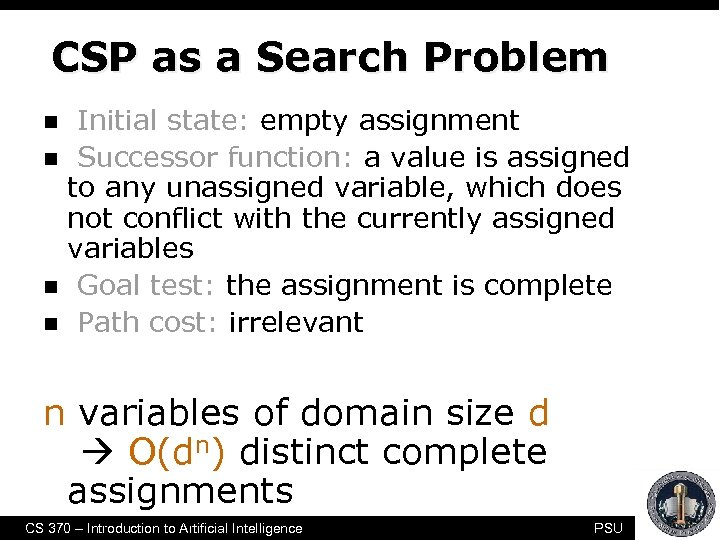CSP as a Search Problem n n Initial state: empty assignment Successor function: a value is assigned to any unassigned variable, which does not conflict with the currently assigned variables Goal test: the assignment is complete Path cost: irrelevant n variables of domain size d O(dn) distinct complete assignments CS 370 – Introduction to Artificial Intelligence PSU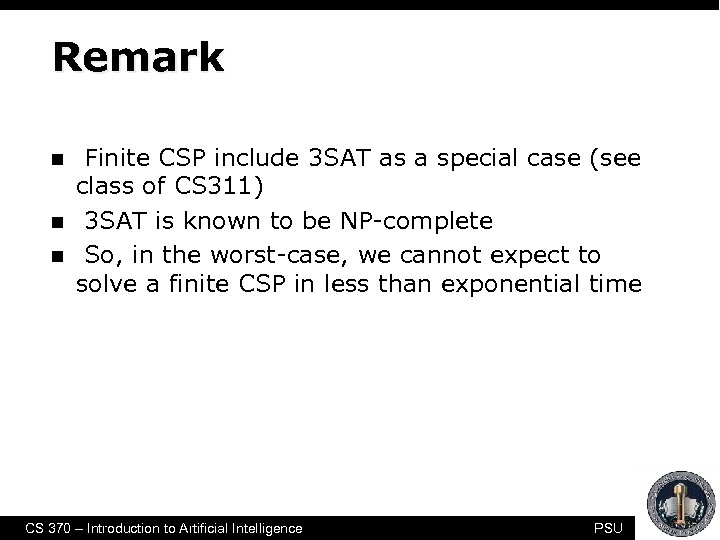Remark n n n Finite CSP include 3 SAT as a special case (see class of CS 311) 3 SAT is known to be NP-complete So, in the worst-case, we cannot expect to solve a finite CSP in less than exponential time CS 370 – Introduction to Artificial Intelligence PSU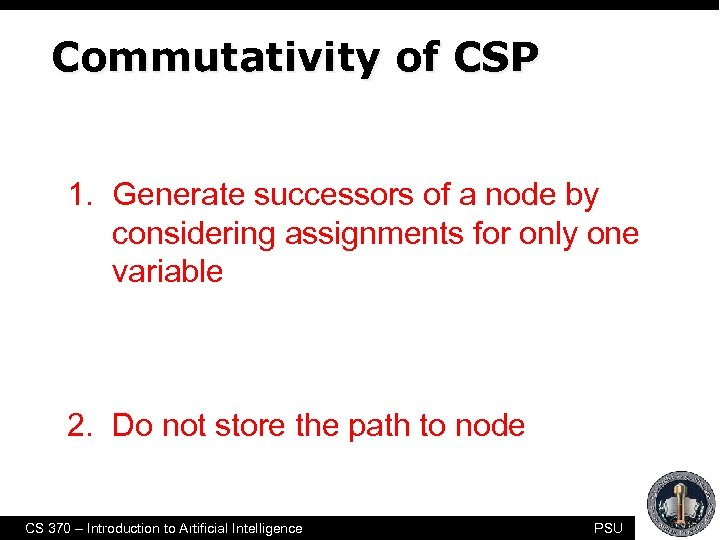Commutativity of CSP 1. Generate successors of a node by considering assignments for only one variable 2. Do not store the path to node CS 370 – Introduction to Artificial Intelligence PSU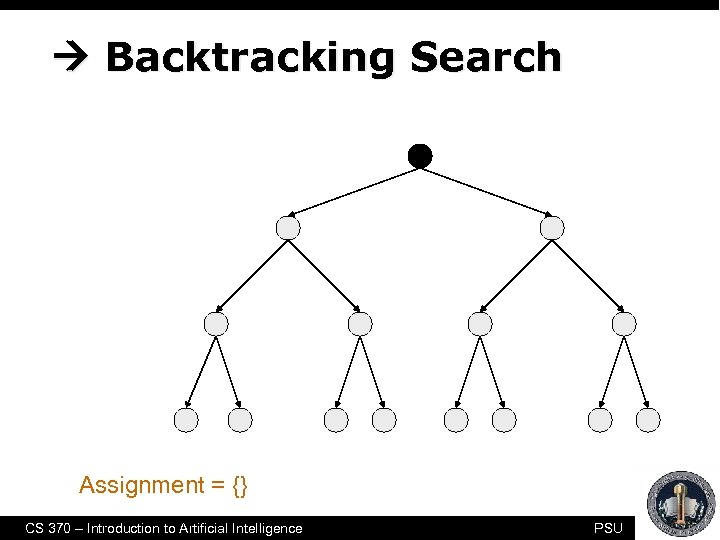Backtracking Search empty assignment 1 st variable 2 nd variable 3 rd variable Assignment = {} CS 370 – Introduction to Artificial Intelligence PSU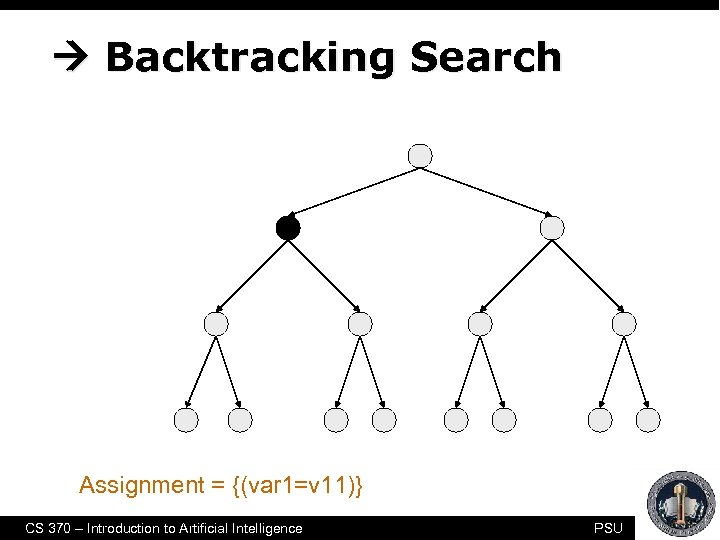Backtracking Search empty assignment 1 st variable 2 nd variable 3 rd variable Assignment = {(var 1=v 11)} CS 370 – Introduction to Artificial Intelligence PSU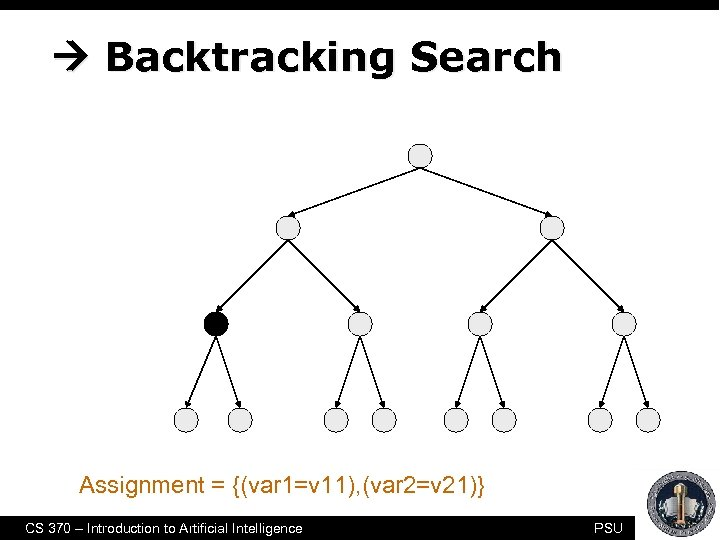Backtracking Search empty assignment 1 st variable 2 nd variable 3 rd variable Assignment = {(var 1=v 11), (var 2=v 21)} CS 370 – Introduction to Artificial Intelligence PSU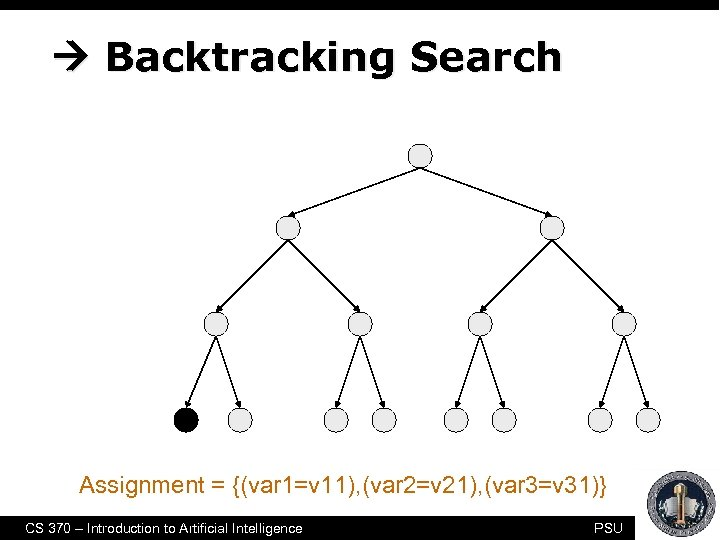Backtracking Search empty assignment 1 st variable 2 nd variable 3 rd variable Assignment = {(var 1=v 11), (var 2=v 21), (var 3=v 31)} CS 370 – Introduction to Artificial Intelligence PSU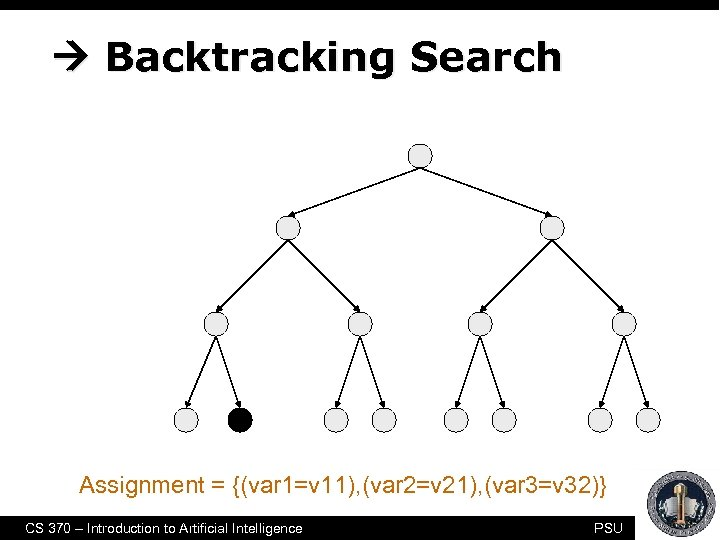Backtracking Search empty assignment 1 st variable 2 nd variable 3 rd variable Assignment = {(var 1=v 11), (var 2=v 21), (var 3=v 32)} CS 370 – Introduction to Artificial Intelligence PSU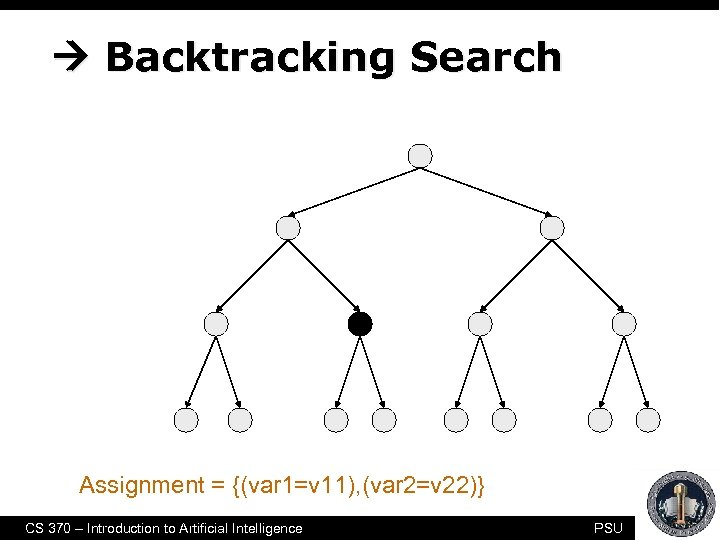Backtracking Search empty assignment 1 st variable 2 nd variable 3 rd variable Assignment = {(var 1=v 11), (var 2=v 22)} CS 370 – Introduction to Artificial Intelligence PSU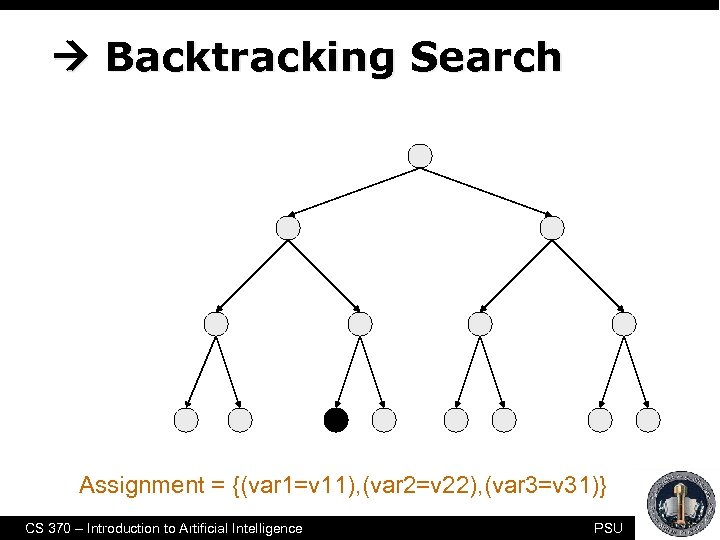Backtracking Search empty assignment 1 st variable 2 nd variable 3 rd variable Assignment = {(var 1=v 11), (var 2=v 22), (var 3=v 31)} CS 370 – Introduction to Artificial Intelligence PSU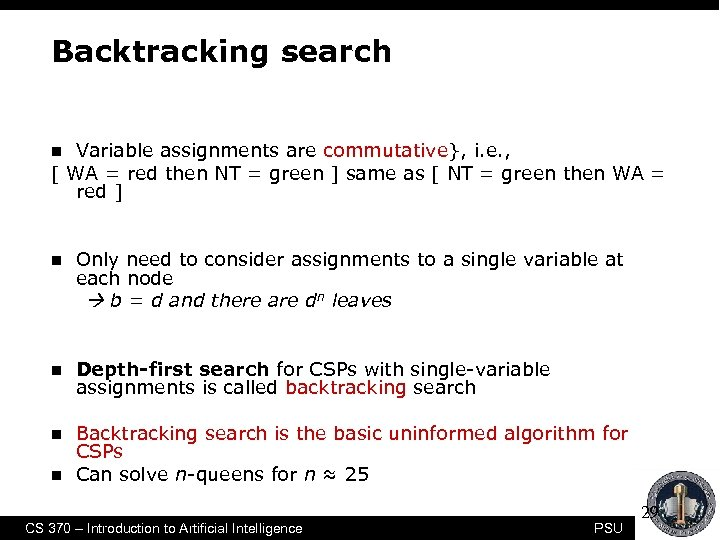Backtracking search Variable assignments are commutative}, i. e. , [ WA = red then NT = green ] same as [ NT = green then WA = red ] n n Only need to consider assignments to a single variable at each node b = d and there are dn leaves n Depth-first search for CSPs with single-variable assignments is called backtracking search n Backtracking search is the basic uninformed algorithm for CSPs Can solve n-queens for n ≈ 25 n CS 370 – Introduction to Artificial Intelligence PSU 29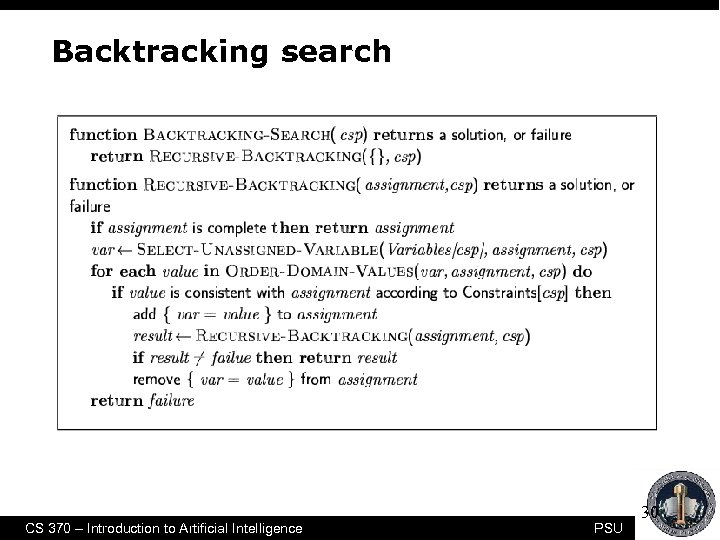Backtracking search CS 370 – Introduction to Artificial Intelligence PSU 30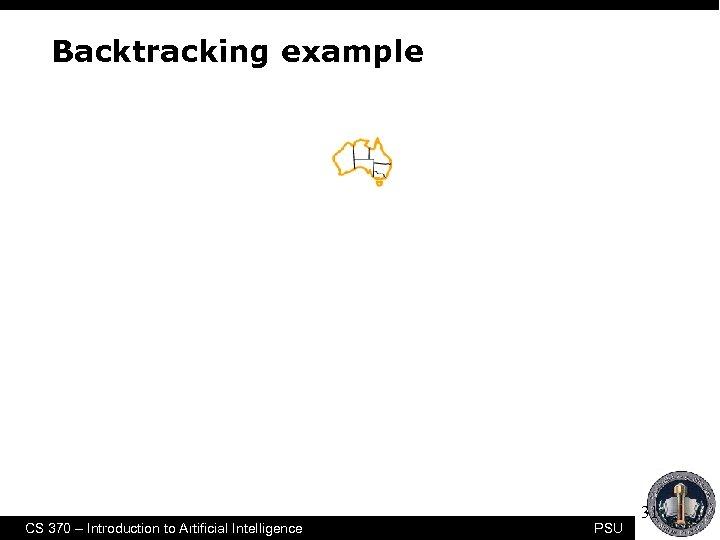Backtracking example CS 370 – Introduction to Artificial Intelligence PSU 31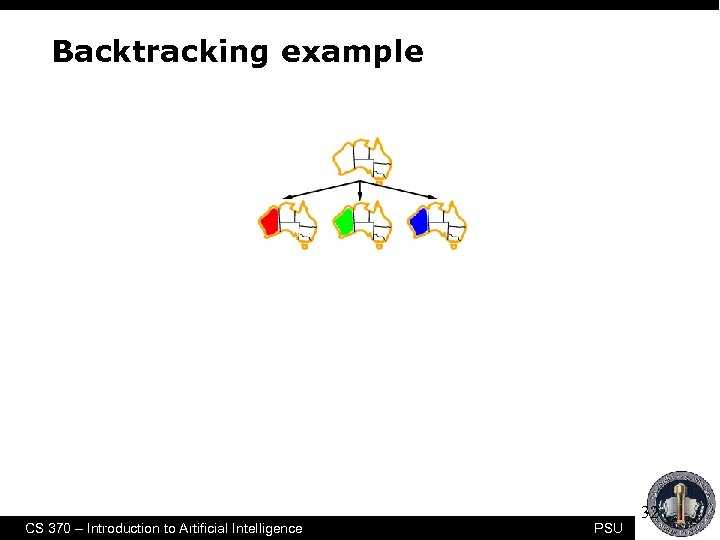Backtracking example CS 370 – Introduction to Artificial Intelligence PSU 32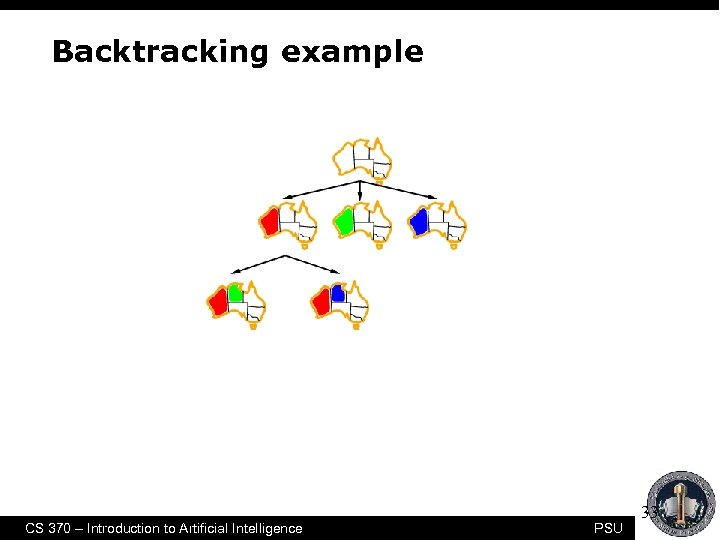Backtracking example CS 370 – Introduction to Artificial Intelligence PSU 33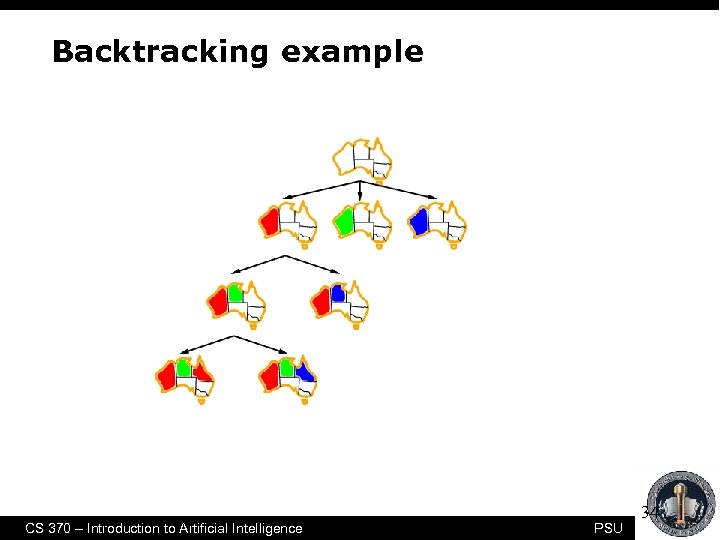Backtracking example CS 370 – Introduction to Artificial Intelligence PSU 34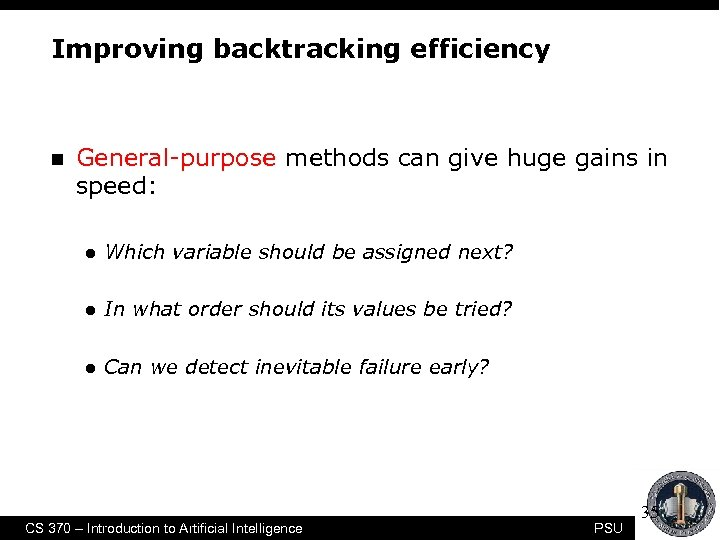Improving backtracking efficiency n General-purpose methods can give huge gains in speed: l Which variable should be assigned next? l In what order should its values be tried? l Can we detect inevitable failure early? CS 370 – Introduction to Artificial Intelligence PSU 35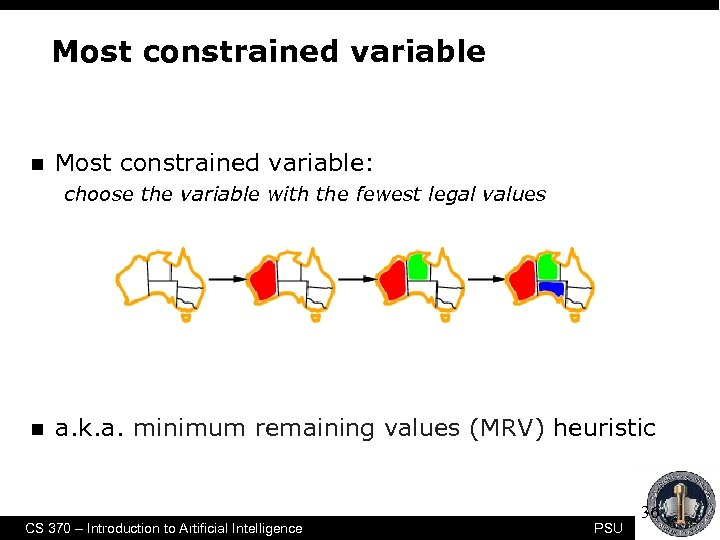Most constrained variable n Most constrained variable: choose the variable with the fewest legal values n a. k. a. minimum remaining values (MRV) heuristic CS 370 – Introduction to Artificial Intelligence PSU 36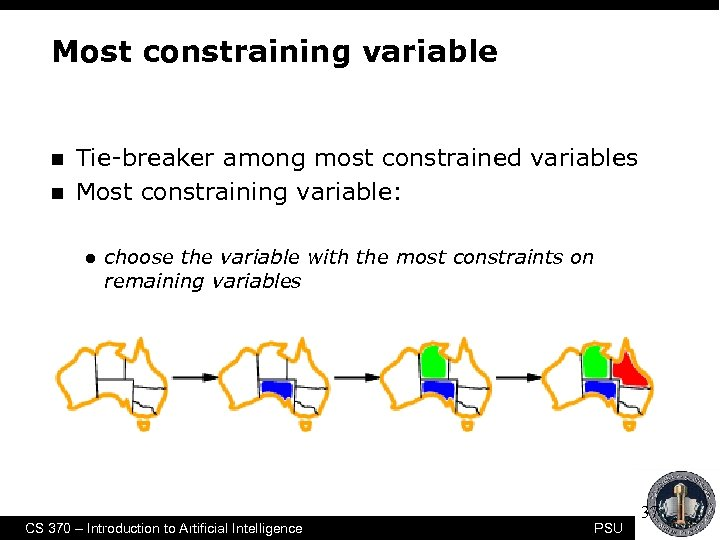Most constraining variable n n Tie-breaker among most constrained variables Most constraining variable: l choose the variable with the most constraints on remaining variables CS 370 – Introduction to Artificial Intelligence PSU 37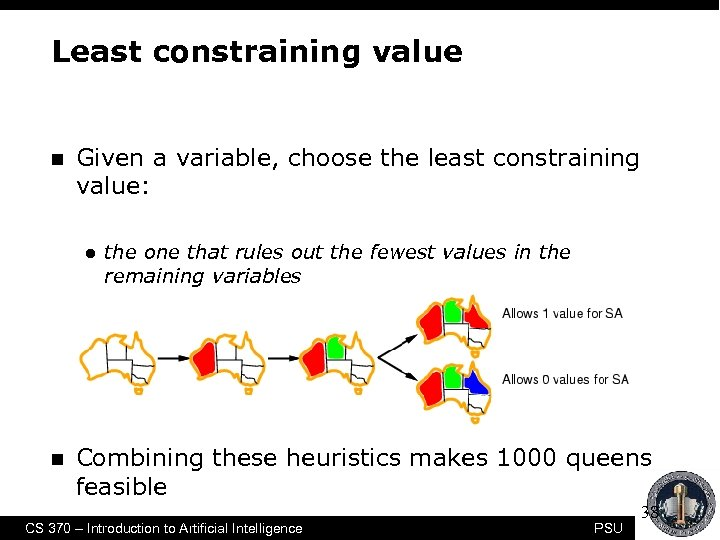Least constraining value n Given a variable, choose the least constraining value: l n the one that rules out the fewest values in the remaining variables Combining these heuristics makes 1000 queens feasible CS 370 – Introduction to Artificial Intelligence PSU 38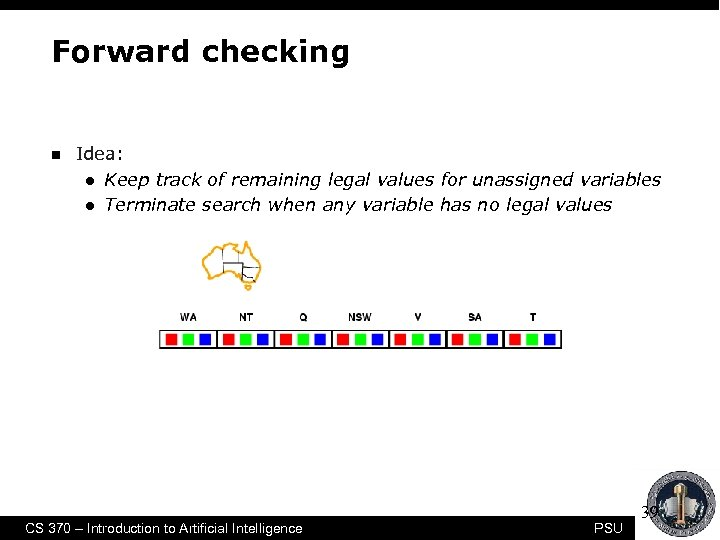Forward checking n Idea: l Keep track of remaining legal values for unassigned variables l Terminate search when any variable has no legal values CS 370 – Introduction to Artificial Intelligence PSU 39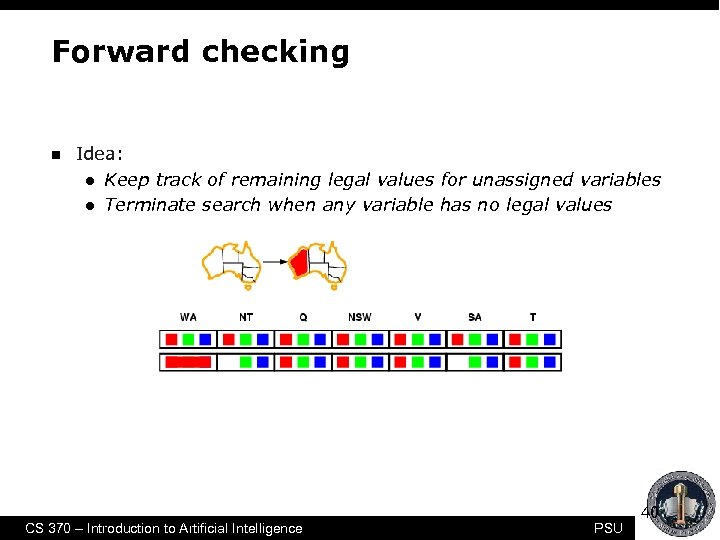Forward checking n Idea: l Keep track of remaining legal values for unassigned variables l Terminate search when any variable has no legal values CS 370 – Introduction to Artificial Intelligence PSU 40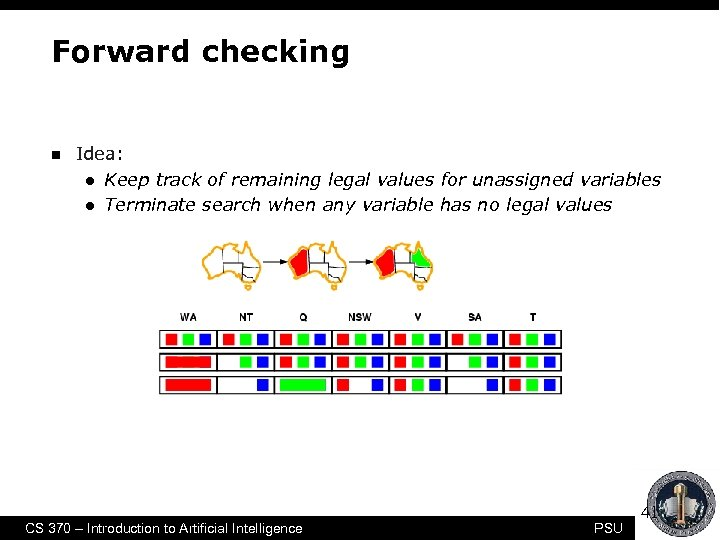Forward checking n Idea: l Keep track of remaining legal values for unassigned variables l Terminate search when any variable has no legal values CS 370 – Introduction to Artificial Intelligence PSU 41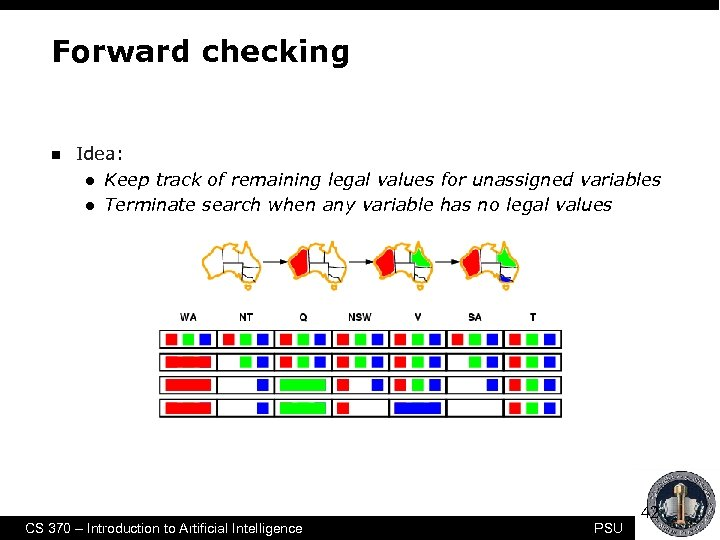Forward checking n Idea: l Keep track of remaining legal values for unassigned variables l Terminate search when any variable has no legal values CS 370 – Introduction to Artificial Intelligence PSU 42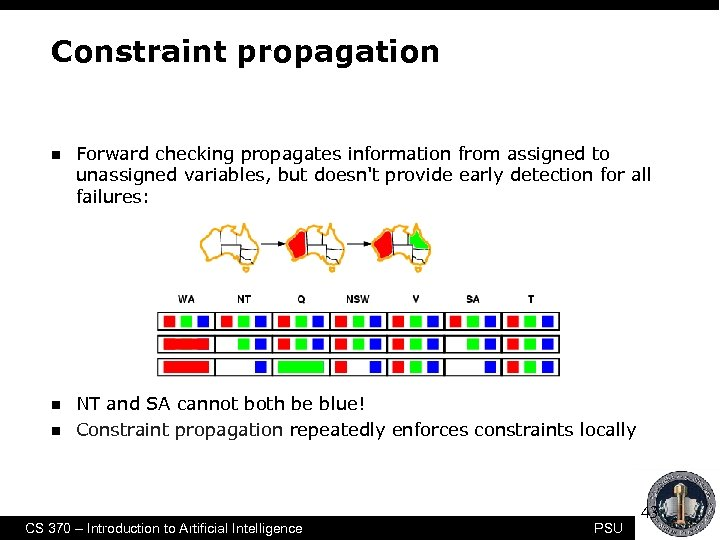Constraint propagation n Forward checking propagates information from assigned to unassigned variables, but doesn't provide early detection for all failures: n NT and SA cannot both be blue! Constraint propagation repeatedly enforces constraints locally n CS 370 – Introduction to Artificial Intelligence PSU 43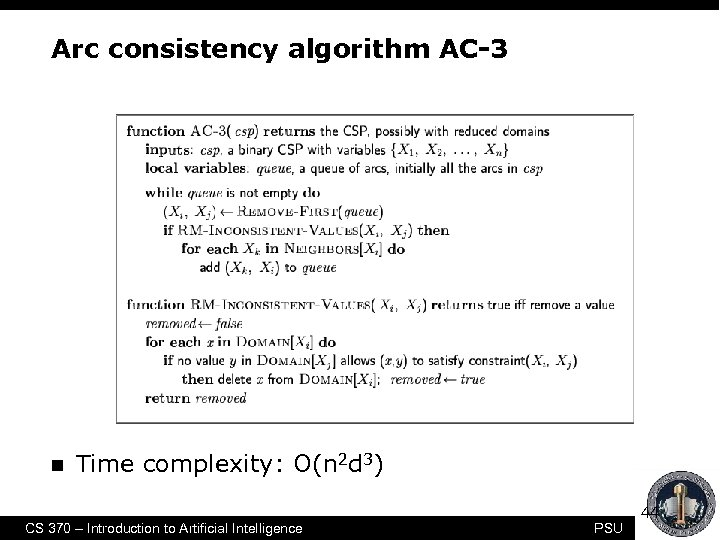Arc consistency algorithm AC-3 n Time complexity: O(n 2 d 3) CS 370 – Introduction to Artificial Intelligence PSU 44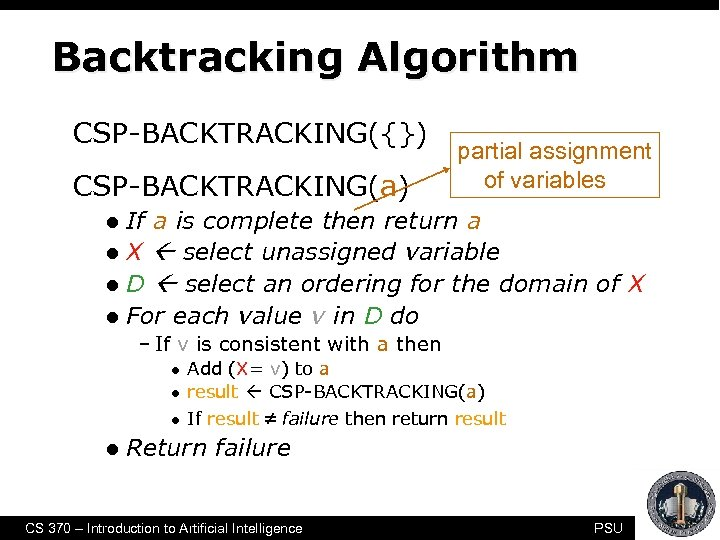Backtracking Algorithm CSP-BACKTRACKING({}) CSP-BACKTRACKING(a) partial assignment of variables If a is complete then return a l X select unassigned variable l D select an ordering for the domain of X l For each value v in D do l – If v is consistent with a then l l Add (X= v) to a result CSP-BACKTRACKING(a) If result failure then return result Return failure CS 370 – Introduction to Artificial Intelligence PSU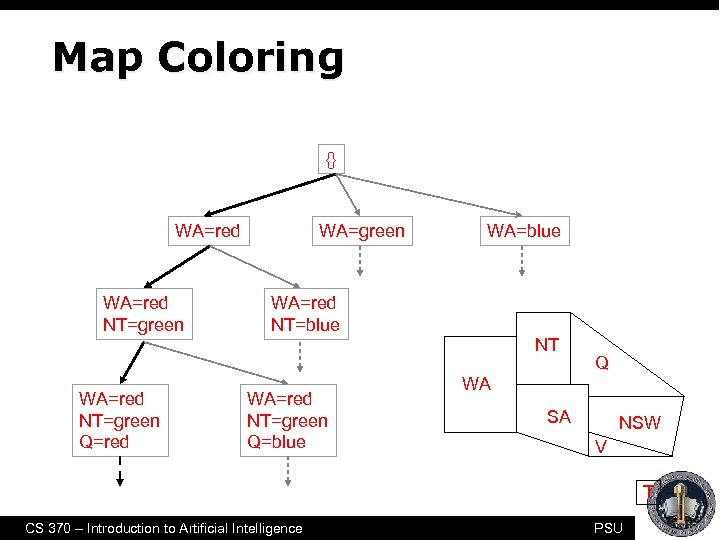Map Coloring {} WA=red NT=green WA=blue WA=red NT=blue NT WA=red NT=green Q=red WA=red NT=green Q=blue Q WA SA NSW V T CS 370 – Introduction to Artificial Intelligence PSU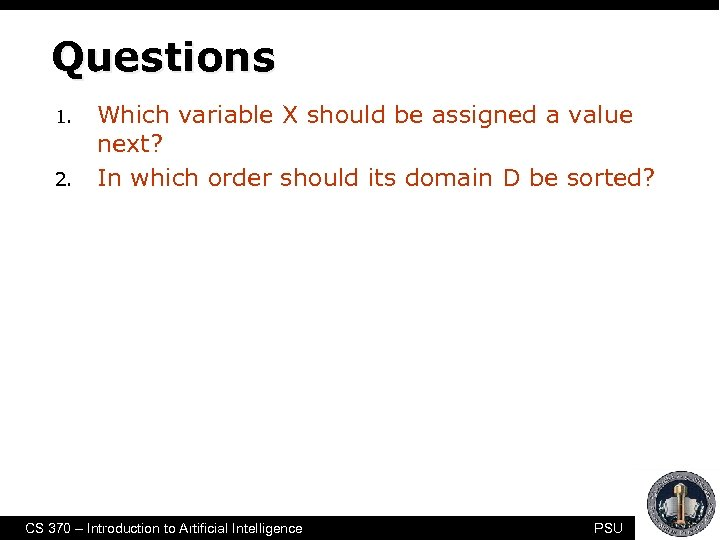Questions 1. 2. Which variable X should be assigned a value next? In which order should its domain D be sorted? CS 370 – Introduction to Artificial Intelligence PSU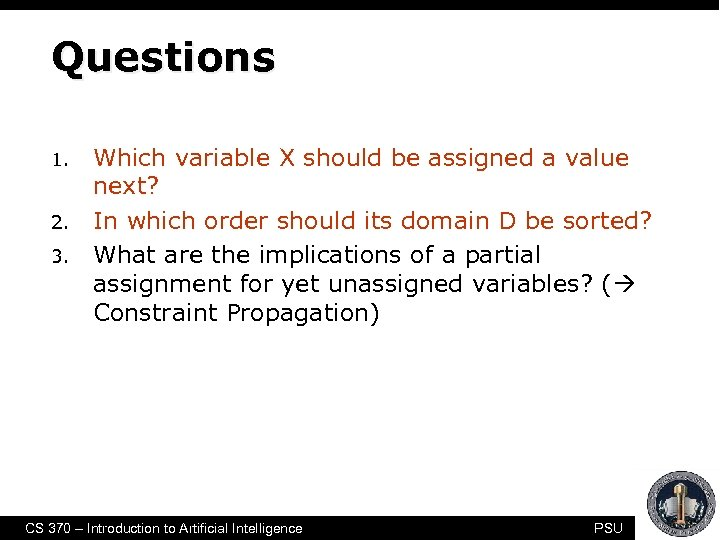Questions 1. 2. 3. Which variable X should be assigned a value next? In which order should its domain D be sorted? What are the implications of a partial assignment for yet unassigned variables? ( Constraint Propagation) CS 370 – Introduction to Artificial Intelligence PSU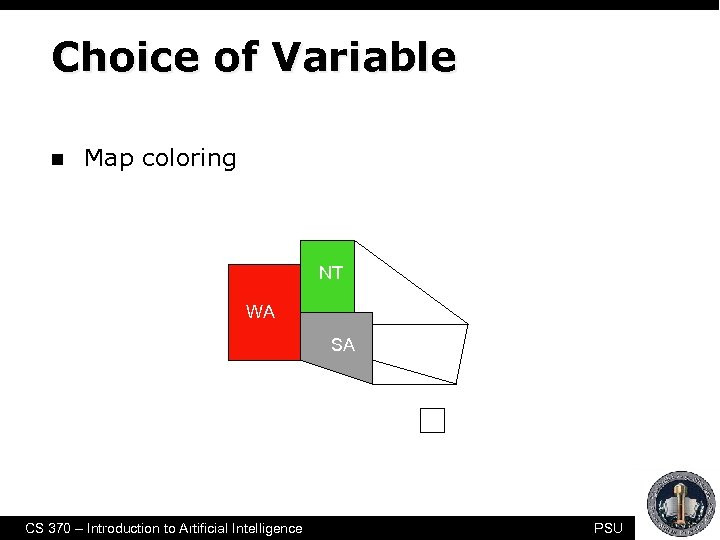Choice of Variable n Map coloring NT Q WA SA NSW V T CS 370 – Introduction to Artificial Intelligence PSU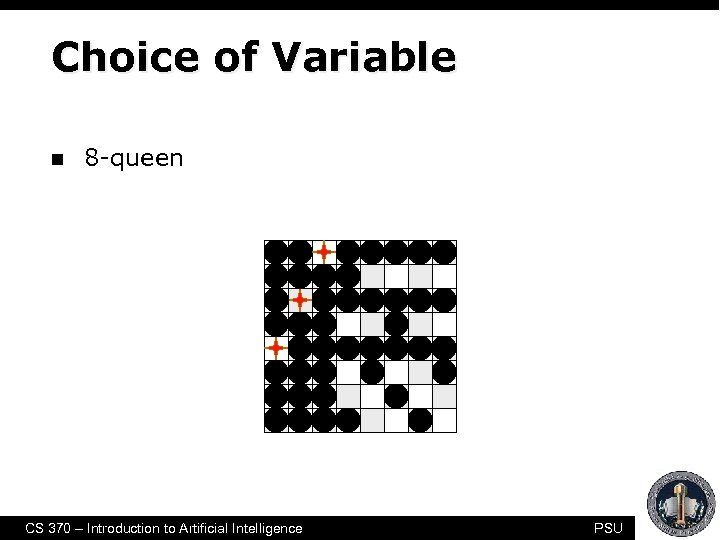Choice of Variable n 8 -queen CS 370 – Introduction to Artificial Intelligence PSU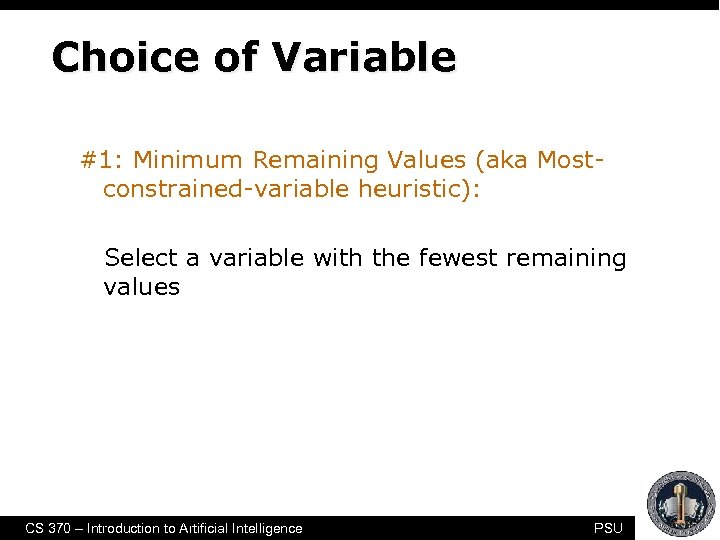Choice of Variable #1: Minimum Remaining Values (aka Mostconstrained-variable heuristic): Select a variable with the fewest remaining values CS 370 – Introduction to Artificial Intelligence PSU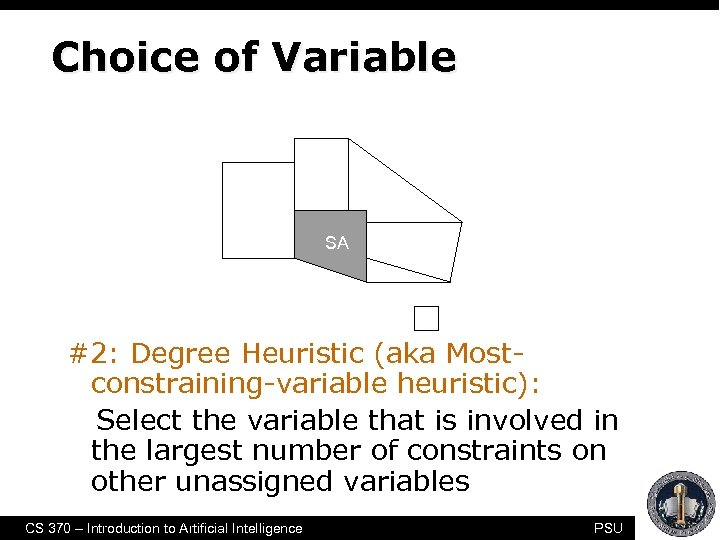Choice of Variable NT Q WA SA NSW V T #2: Degree Heuristic (aka Mostconstraining-variable heuristic): Select the variable that is involved in the largest number of constraints on other unassigned variables CS 370 – Introduction to Artificial Intelligence PSU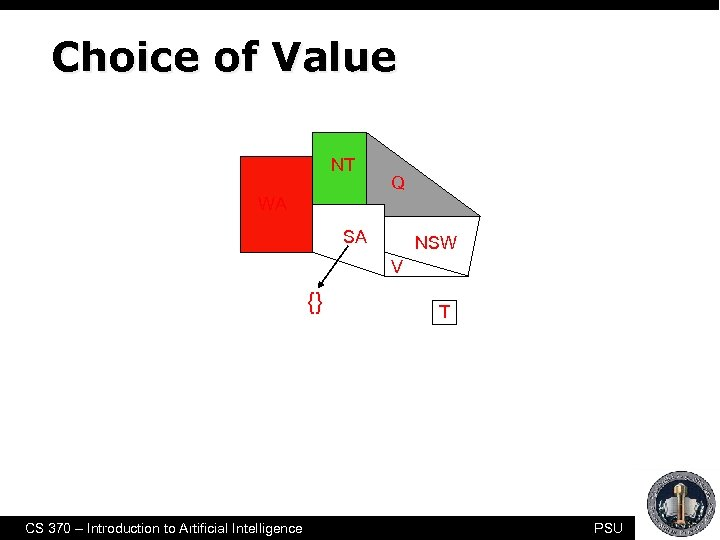Choice of Value NT Q WA SA NSW V {} CS 370 – Introduction to Artificial Intelligence T PSU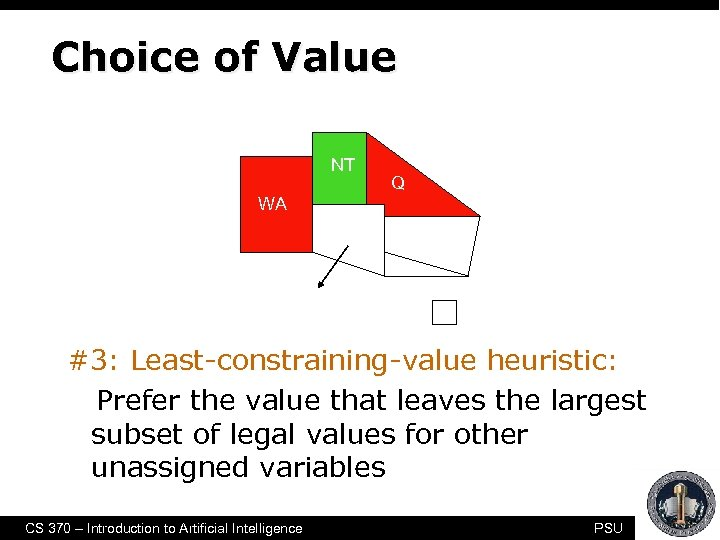Choice of Value NT Q WA SA NSW V {blue} T #3: Least-constraining-value heuristic: Prefer the value that leaves the largest subset of legal values for other unassigned variables CS 370 – Introduction to Artificial Intelligence PSU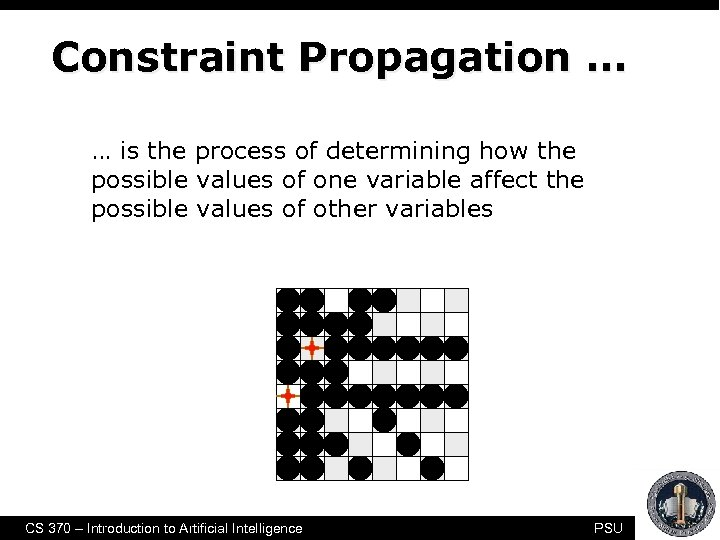Constraint Propagation … … is the process of determining how the possible values of one variable affect the possible values of other variables CS 370 – Introduction to Artificial Intelligence PSU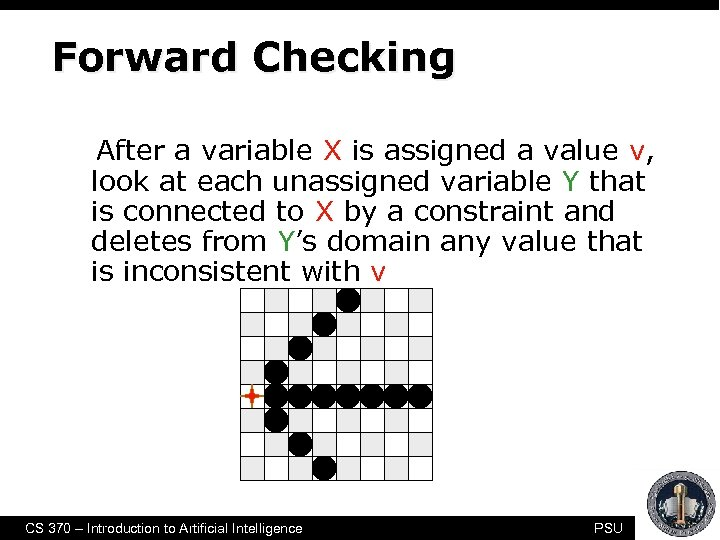Forward Checking After a variable X is assigned a value v, look at each unassigned variable Y that is connected to X by a constraint and deletes from Y’s domain any value that is inconsistent with v CS 370 – Introduction to Artificial Intelligence PSU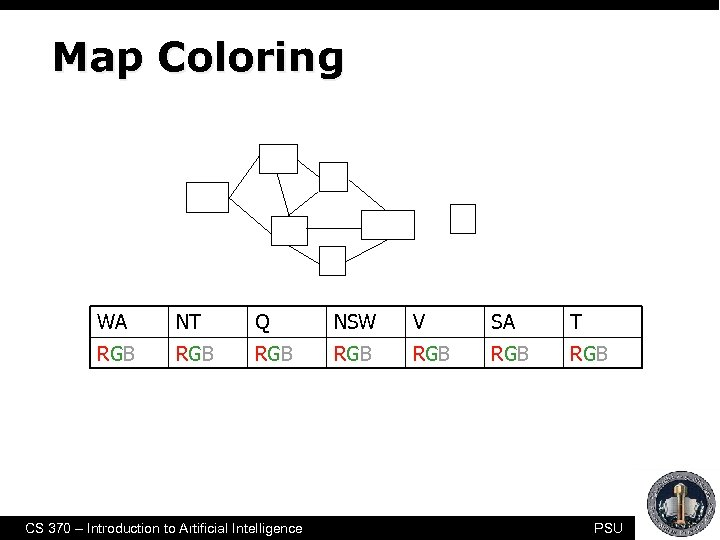Map Coloring NT Q WA T NSW SA V WA NT Q NSW V SA T RGB RGB CS 370 – Introduction to Artificial Intelligence PSU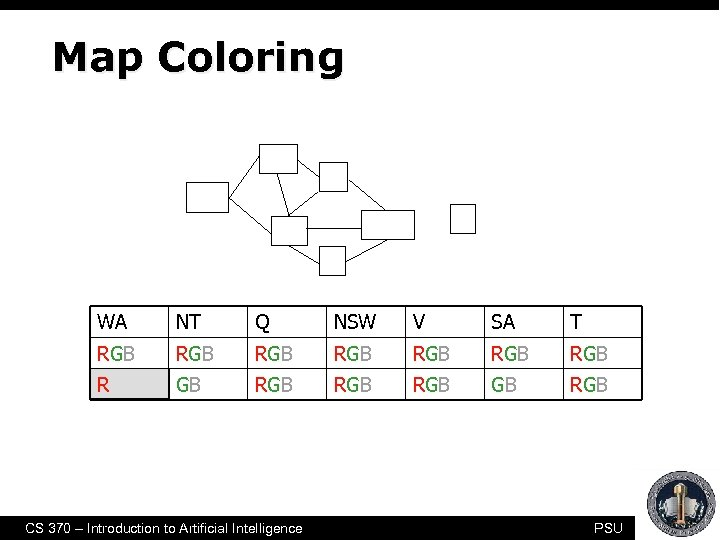Map Coloring NT Q WA T NSW SA V WA NT Q NSW V SA T RGB RGB R GB RGB RGB CS 370 – Introduction to Artificial Intelligence PSU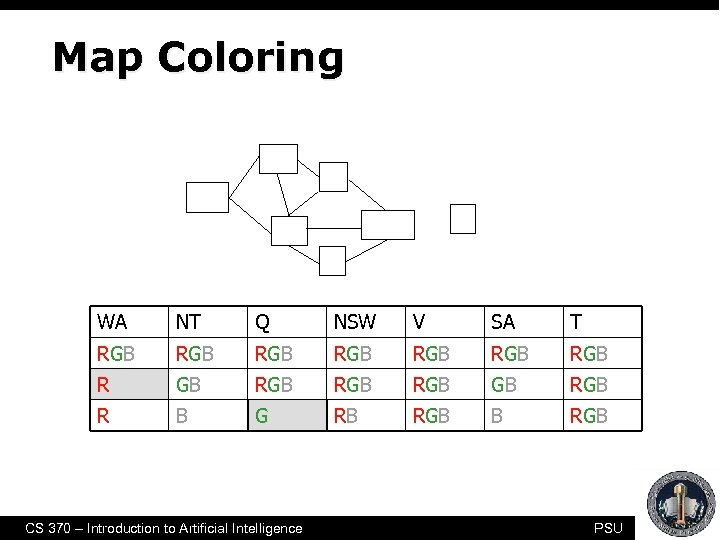Map Coloring NT Q WA T NSW SA V WA NT Q NSW V SA T RGB RGB R GB RGB RGB R B G RB RGB CS 370 – Introduction to Artificial Intelligence PSU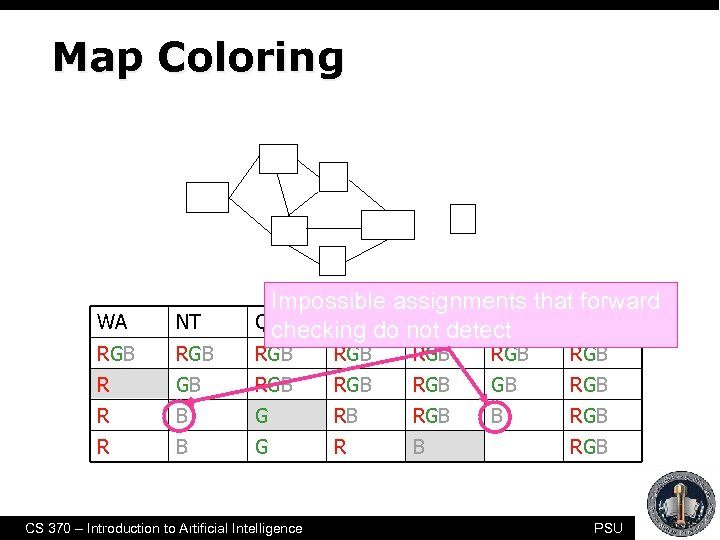Map Coloring NT Q WA T NSW SA V WA NT RGB R Impossible assignments that forward Q checking do not detect NSW V SA T RGB RGB RGB R B G RB RGB R B G R B CS 370 – Introduction to Artificial Intelligence RGB PSU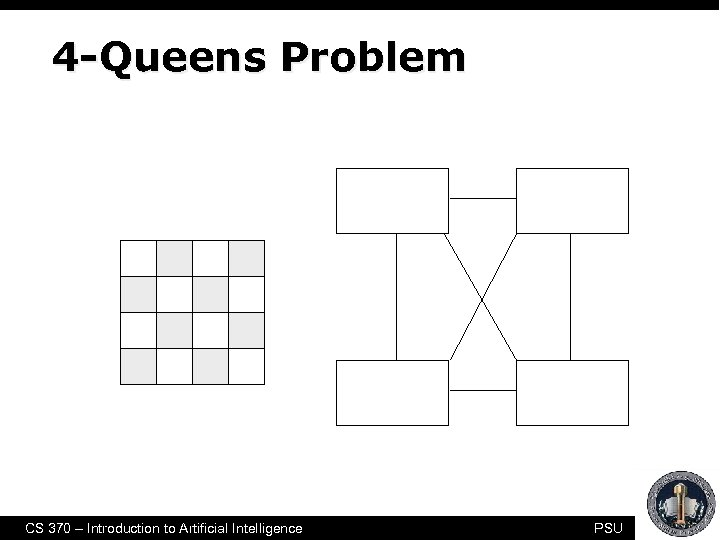4 -Queens Problem 1 2 3 4 X 1 {1, 2, 3, 4} X 2 {1, 2, 3, 4} X 3 {1, 2, 3, 4} X 4 {1, 2, 3, 4} 1 2 3 4 CS 370 – Introduction to Artificial Intelligence PSU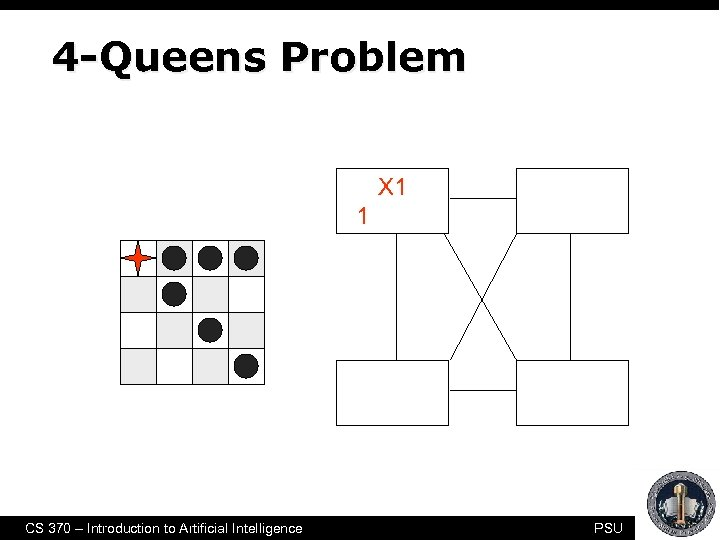4 -Queens Problem 1 2 3 4 X 1 {1, 2, 3, 4} X 2 {1, 2, 3, 4} X 3 {1, 2, 3, 4} X 4 {1, 2, 3, 4} 1 2 3 4 CS 370 – Introduction to Artificial Intelligence PSU4 -Queens Problem 1 2 3 4 X 1 {1, 2, 3, 4} X 2 {1, 2, 3, 4} X 3 {1, 2, 3, 4} X 4 {1, 2, 3, 4} 1 2 3 4 CS 370 – Introduction to Artificial Intelligence PSU4 -Queens Problem 1 2 3 4 X 1 {1, 2, 3, 4} X 2 {1, 2, 3, 4} X 3 {1, 2, 3, 4} X 4 {1, 2, 3, 4} 1 2 3 4 CS 370 – Introduction to Artificial Intelligence PSU4 -Queens Problem 1 2 3 4 X 1 {1, 2, 3, 4} X 2 {1, 2, 3, 4} X 3 {1, 2, 3, 4} X 4 {1, 2, 3, 4} 1 2 3 4 CS 370 – Introduction to Artificial Intelligence PSU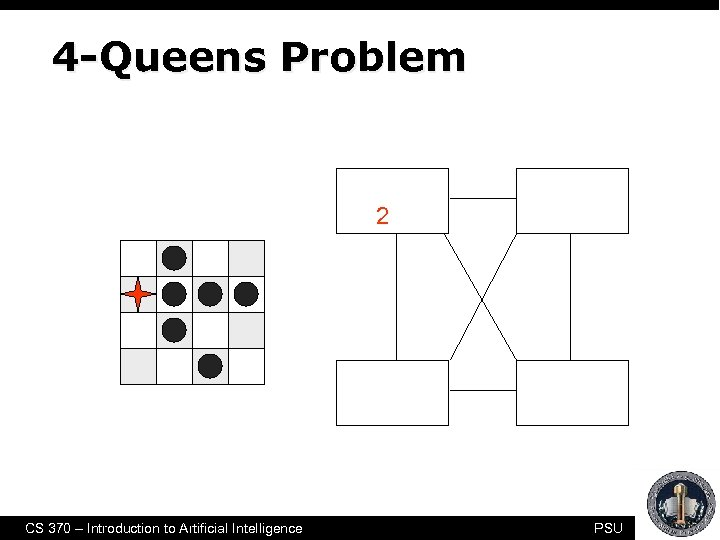4 -Queens Problem 1 2 3 4 X 1 {1, 2, 3, 4} X 2 {1, 2, 3, 4} X 3 {1, 2, 3, 4} X 4 {1, 2, 3, 4} 1 2 3 4 CS 370 – Introduction to Artificial Intelligence PSU4 -Queens Problem 1 2 3 4 X 1 {1, 2, 3, 4} X 2 {1, 2, 3, 4} X 3 {1, 2, 3, 4} X 4 {1, 2, 3, 4} 1 2 3 4 CS 370 – Introduction to Artificial Intelligence PSU4 -Queens Problem 1 2 3 4 X 1 {1, 2, 3, 4} X 2 {1, 2, 3, 4} X 3 {1, 2, 3, 4} X 4 {1, 2, 3, 4} 1 2 3 4 CS 370 – Introduction to Artificial Intelligence PSU4 -Queens Problem 1 2 3 4 X 1 {1, 2, 3, 4} X 2 {1, 2, 3, 4} X 3 {1, 2, 3, 4} X 4 {1, 2, 3, 4} 1 2 3 4 CS 370 – Introduction to Artificial Intelligence PSU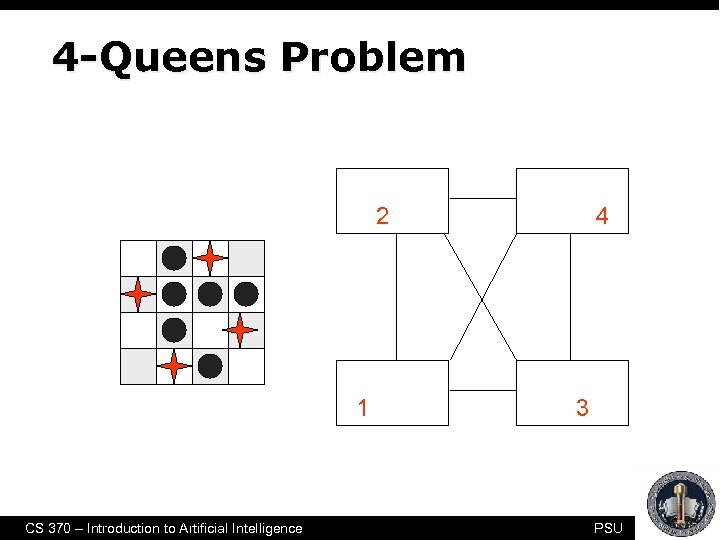4 -Queens Problem 1 2 3 4 X 1 {1, 2, 3, 4} X 2 {1, 2, 3, 4} X 3 {1, 2, 3, 4} X 4 {1, 2, 3, 4} 1 2 3 4 CS 370 – Introduction to Artificial Intelligence PSU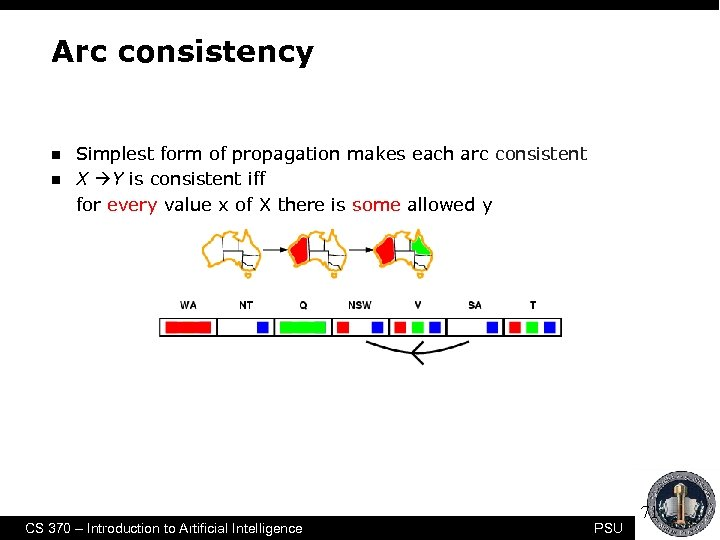Arc consistency n n Simplest form of propagation makes each arc consistent X Y is consistent iff for every value x of X there is some allowed y CS 370 – Introduction to Artificial Intelligence PSU 71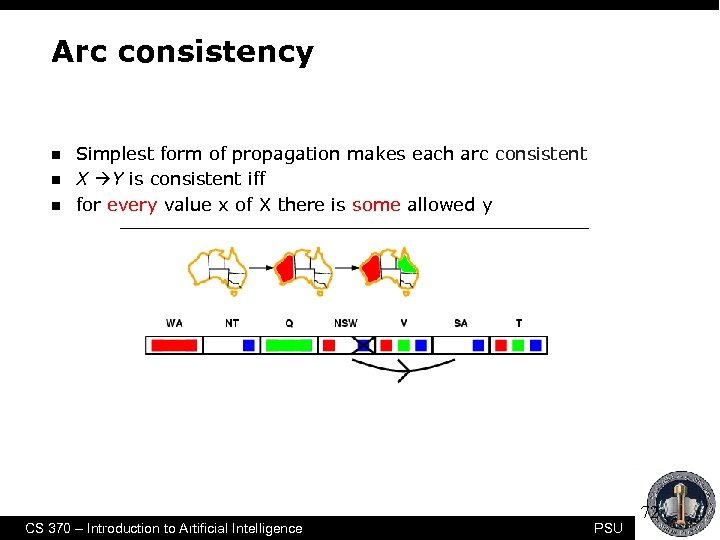Arc consistency n n n Simplest form of propagation makes each arc consistent X Y is consistent iff for every value x of X there is some allowed y CS 370 – Introduction to Artificial Intelligence PSU 72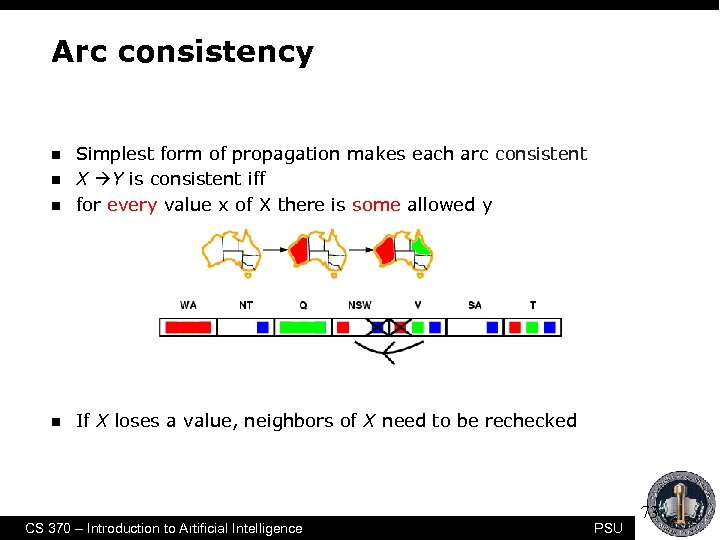Arc consistency n Simplest form of propagation makes each arc consistent X Y is consistent iff for every value x of X there is some allowed y n If X loses a value, neighbors of X need to be rechecked n n CS 370 – Introduction to Artificial Intelligence PSU 73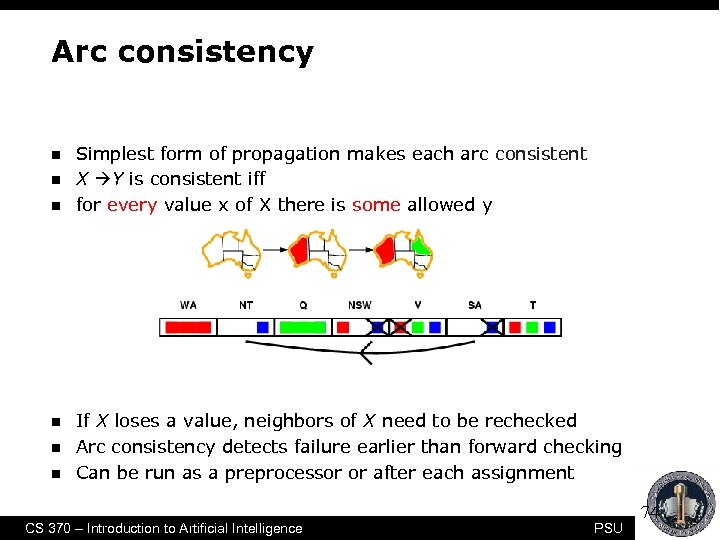Arc consistency n n n Simplest form of propagation makes each arc consistent X Y is consistent iff for every value x of X there is some allowed y If X loses a value, neighbors of X need to be rechecked Arc consistency detects failure earlier than forward checking Can be run as a preprocessor or after each assignment CS 370 – Introduction to Artificial Intelligence PSU 74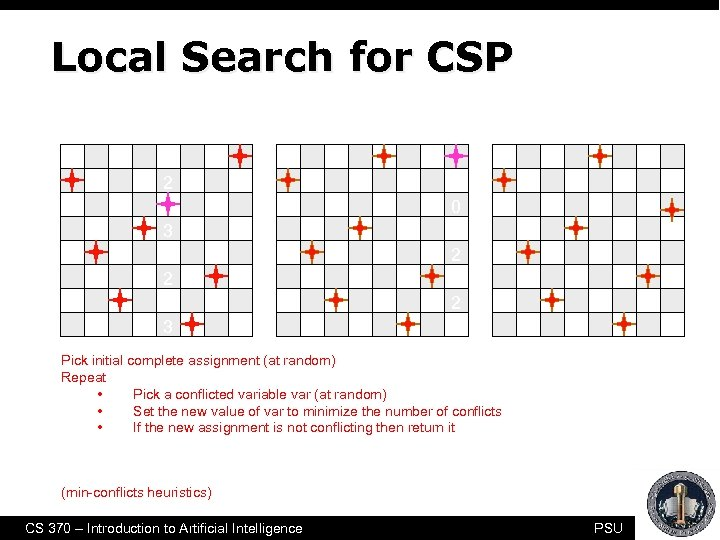Local Search for CSP 1 2 3 3 2 2 3 2 0 2 2 2 Pick initial complete assignment (at random) Repeat • Pick a conflicted variable var (at random) • Set the new value of var to minimize the number of conflicts • If the new assignment is not conflicting then return it (min-conflicts heuristics) CS 370 – Introduction to Artificial Intelligence PSU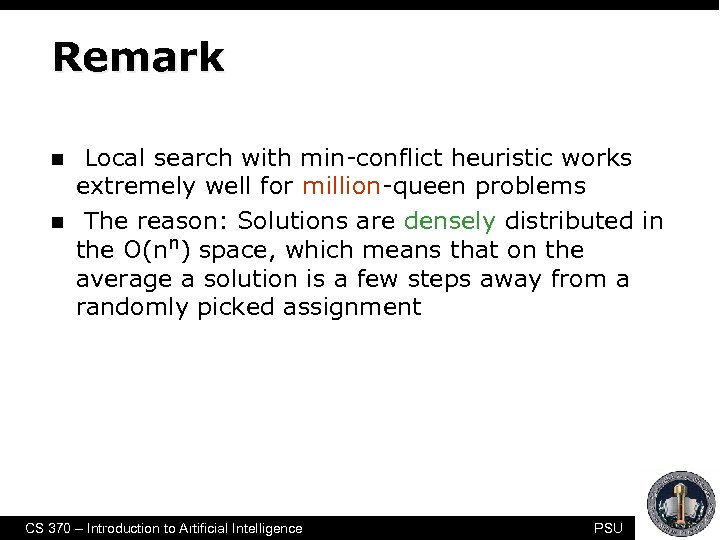Remark n n Local search with min-conflict heuristic works extremely well for million-queen problems The reason: Solutions are densely distributed in the O(nn) space, which means that on the average a solution is a few steps away from a randomly picked assignment CS 370 – Introduction to Artificial Intelligence PSU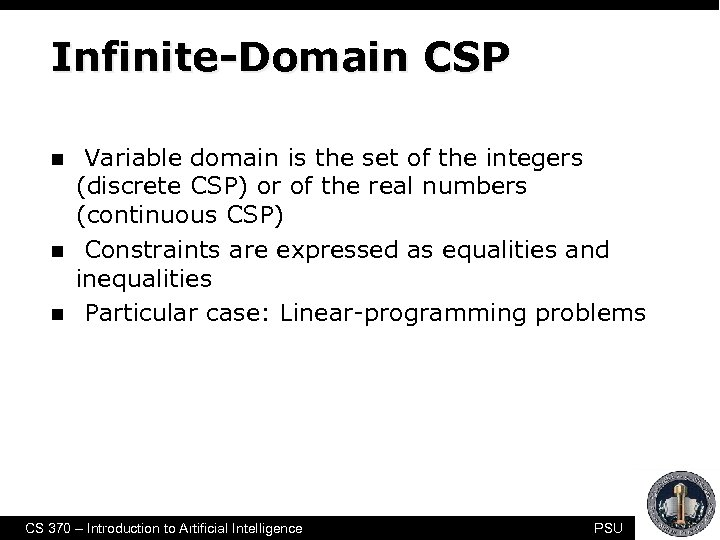Infinite-Domain CSP n n n Variable domain is the set of the integers (discrete CSP) or of the real numbers (continuous CSP) Constraints are expressed as equalities and inequalities Particular case: Linear-programming problems CS 370 – Introduction to Artificial Intelligence PSU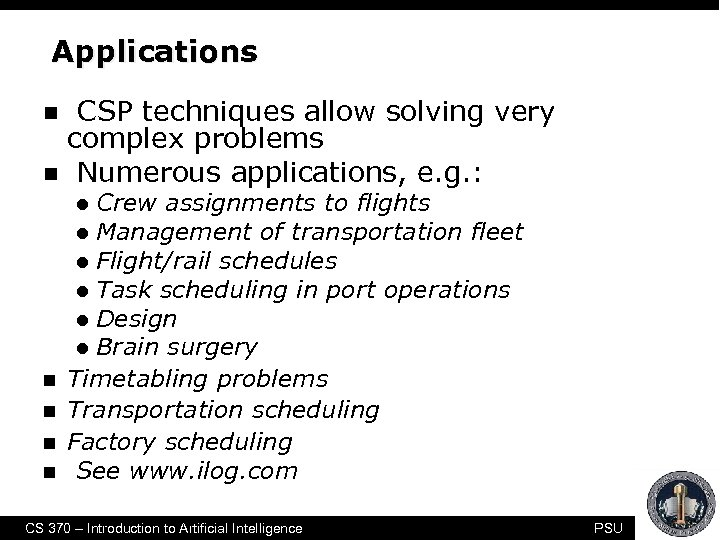Applications n n CSP techniques allow solving very complex problems Numerous applications, e. g. : Crew assignments to flights l Management of transportation fleet l Flight/rail schedules l Task scheduling in port operations l Design l Brain surgery Timetabling problems Transportation scheduling Factory scheduling See www. ilog. com l n n CS 370 – Introduction to Artificial Intelligence PSUStereotaxic Brain Surgery CS 370 – Introduction to Artificial Intelligence PSU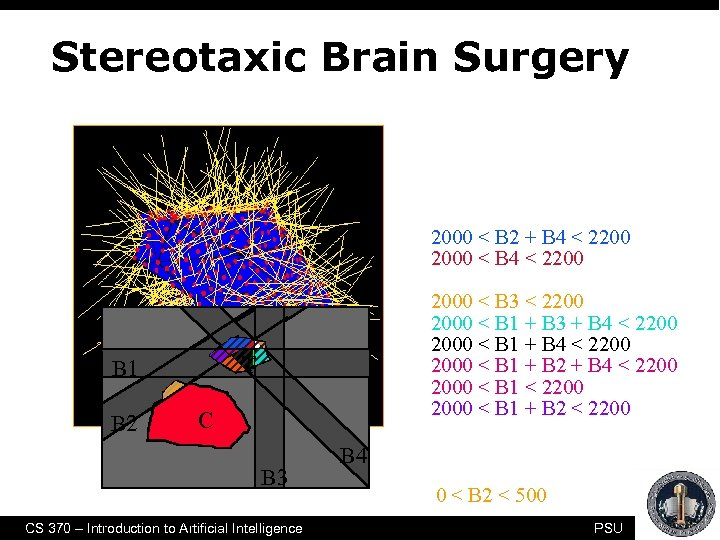Stereotaxic Brain Surgery • • 0 < Critical < 500 0 < B 2 < 500 T B 1 B 2 2000 < Tumor < 2200 2000 < B 2 + B 4 < 2200 2000 < B 3 < 2200 2000 < B 1 + B 3 + B 4 < 2200 2000 < B 1 + B 2 < 2200 C B 3 CS 370 – Introduction to Artificial Intelligence B 4 PSU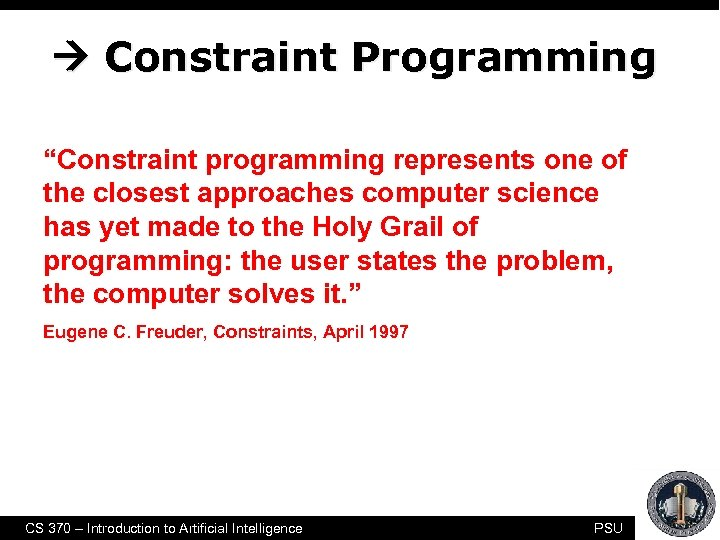Constraint Programming “Constraint programming represents one of the closest approaches computer science has yet made to the Holy Grail of programming: the user states the problem, the computer solves it. ” Eugene C. Freuder, Constraints, April 1997 CS 370 – Introduction to Artificial Intelligence PSU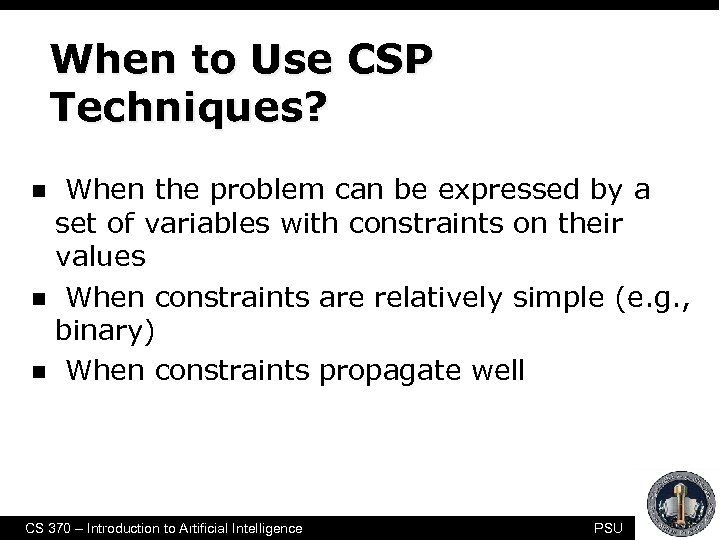When to Use CSP Techniques? n n n When the problem can be expressed by a set of variables with constraints on their values When constraints are relatively simple (e. g. , binary) When constraints propagate well CS 370 – Introduction to Artificial Intelligence PSU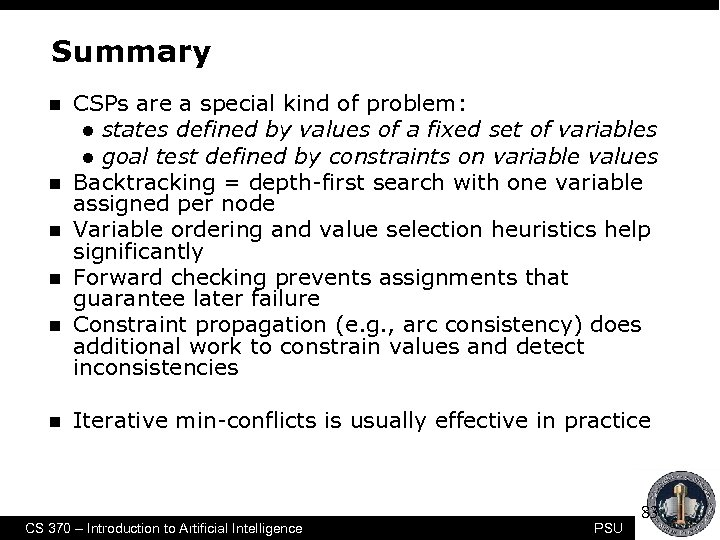Summary n n n CSPs are a special kind of problem: l states defined by values of a fixed set of variables l goal test defined by constraints on variable values Backtracking = depth-first search with one variable assigned per node Variable ordering and value selection heuristics help significantly Forward checking prevents assignments that guarantee later failure Constraint propagation (e. g. , arc consistency) does additional work to constrain values and detect inconsistencies Iterative min-conflicts is usually effective in practice CS 370 – Introduction to Artificial Intelligence PSU 83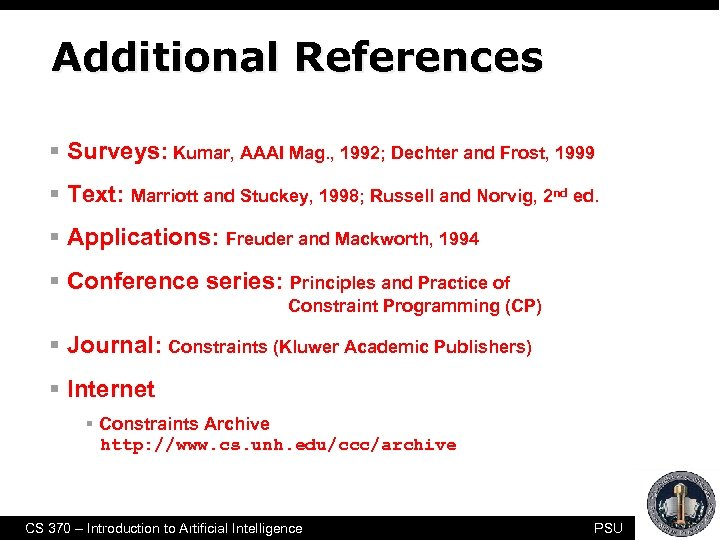Additional References § Surveys: Kumar, AAAI Mag. , 1992; Dechter and Frost, 1999 § Text: Marriott and Stuckey, 1998; Russell and Norvig, 2 nd ed. § Applications: Freuder and Mackworth, 1994 § Conference series: Principles and Practice of Constraint Programming (CP) § Journal: Constraints (Kluwer Academic Publishers) § Internet § Constraints Archive http: //www. cs. unh. edu/ccc/archive CS 370 – Introduction to Artificial Intelligence PSU